# Concise Selina Solutions for Class 9 Maths Chapter 24 - Solution Of Right Triangles

Selina Solutions for Class 9 Maths Chapter 24, Solution Of Right Triangles, are provided here. It is important for students to understand the concepts taught in Class 9 as they are continued in class 10 syllabus as well. To score good marks in Class 9 Maths examination, it is advised to solve the entire questions provided in each exercise across all the chapters in the book by Selina publication. The Selina solutions for Class 9 Maths helps students in understanding all the concepts in a better way. Download pdf of Class 9 Maths Chapter 24 Selina Solutions from the given link.

### Download PDF of Selina Solutions for Class 9 Maths Chapter 24:-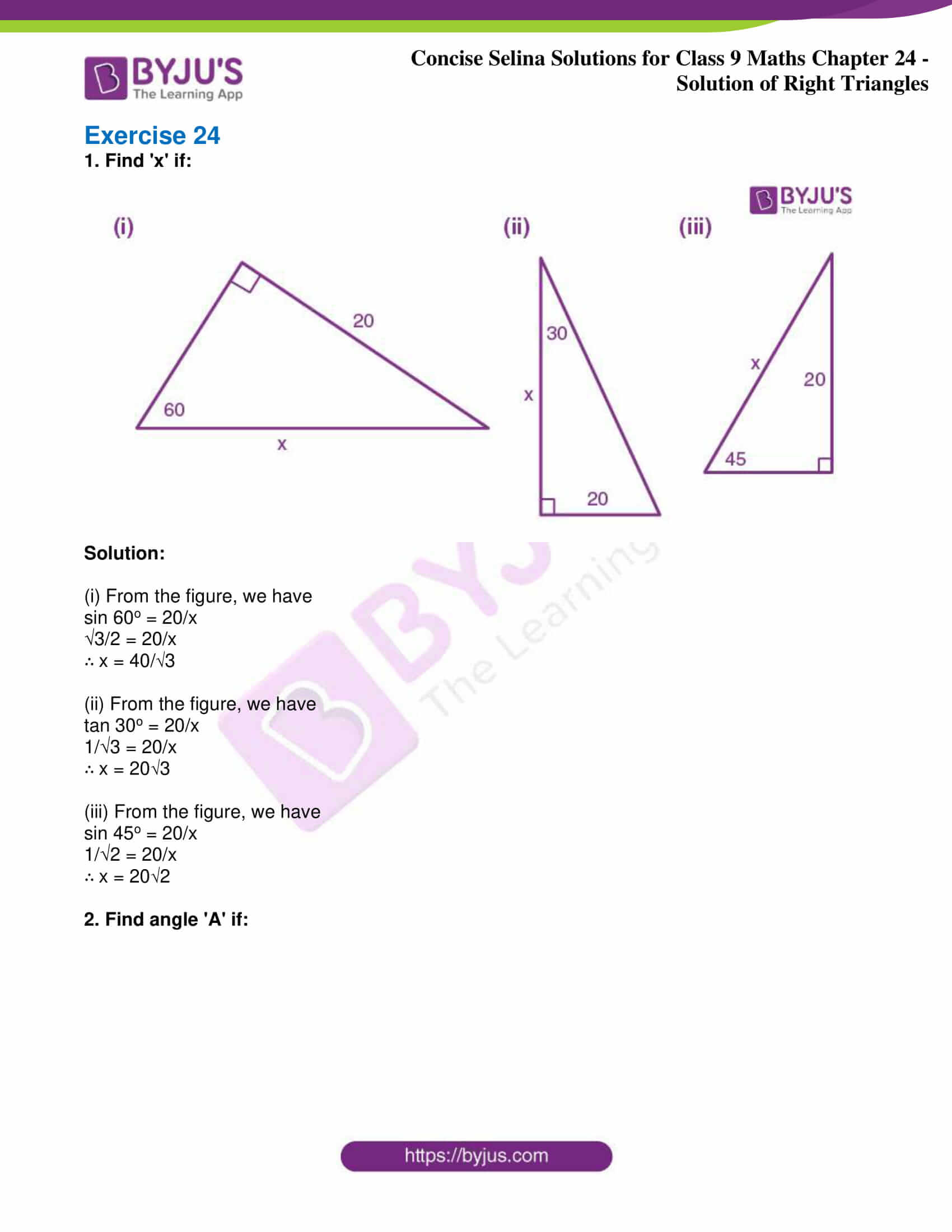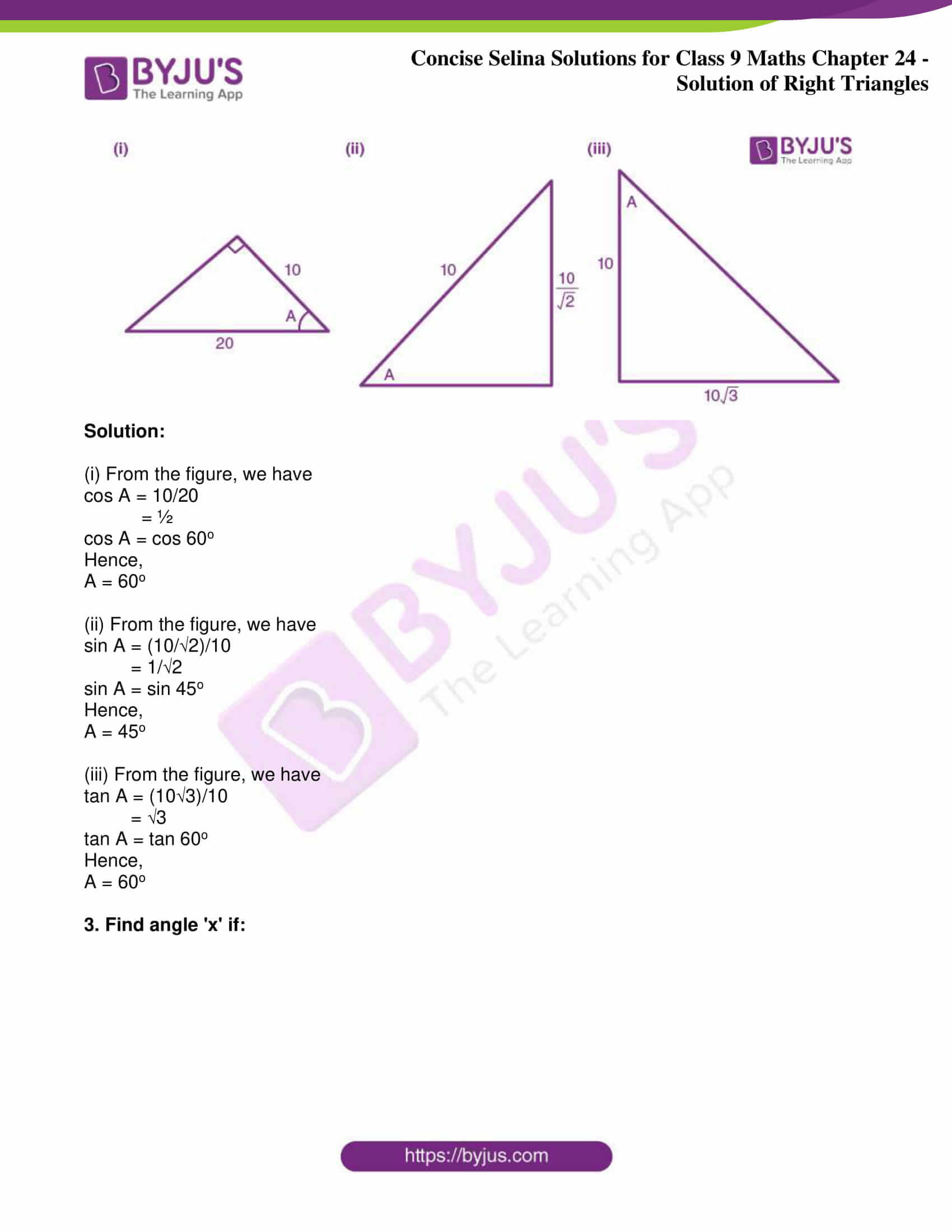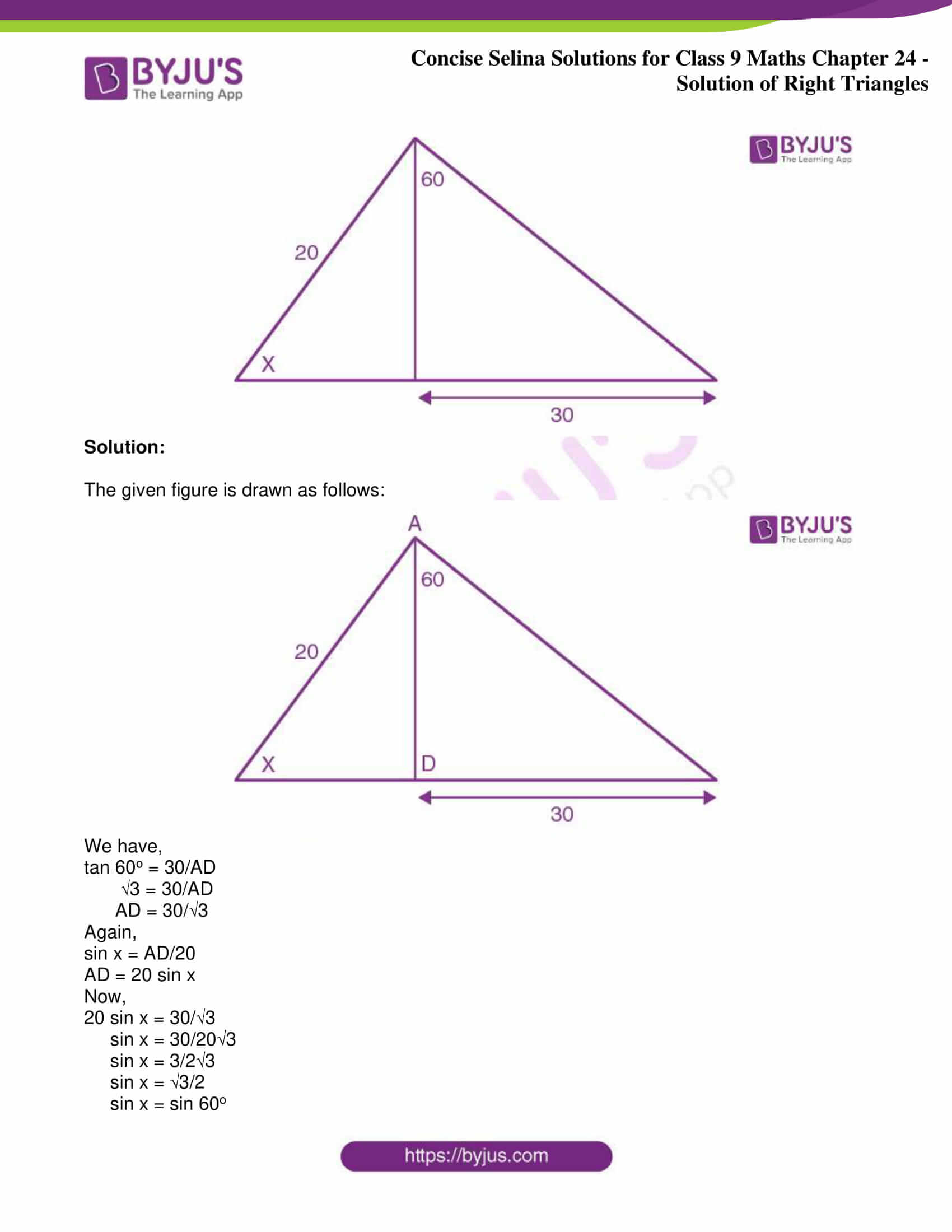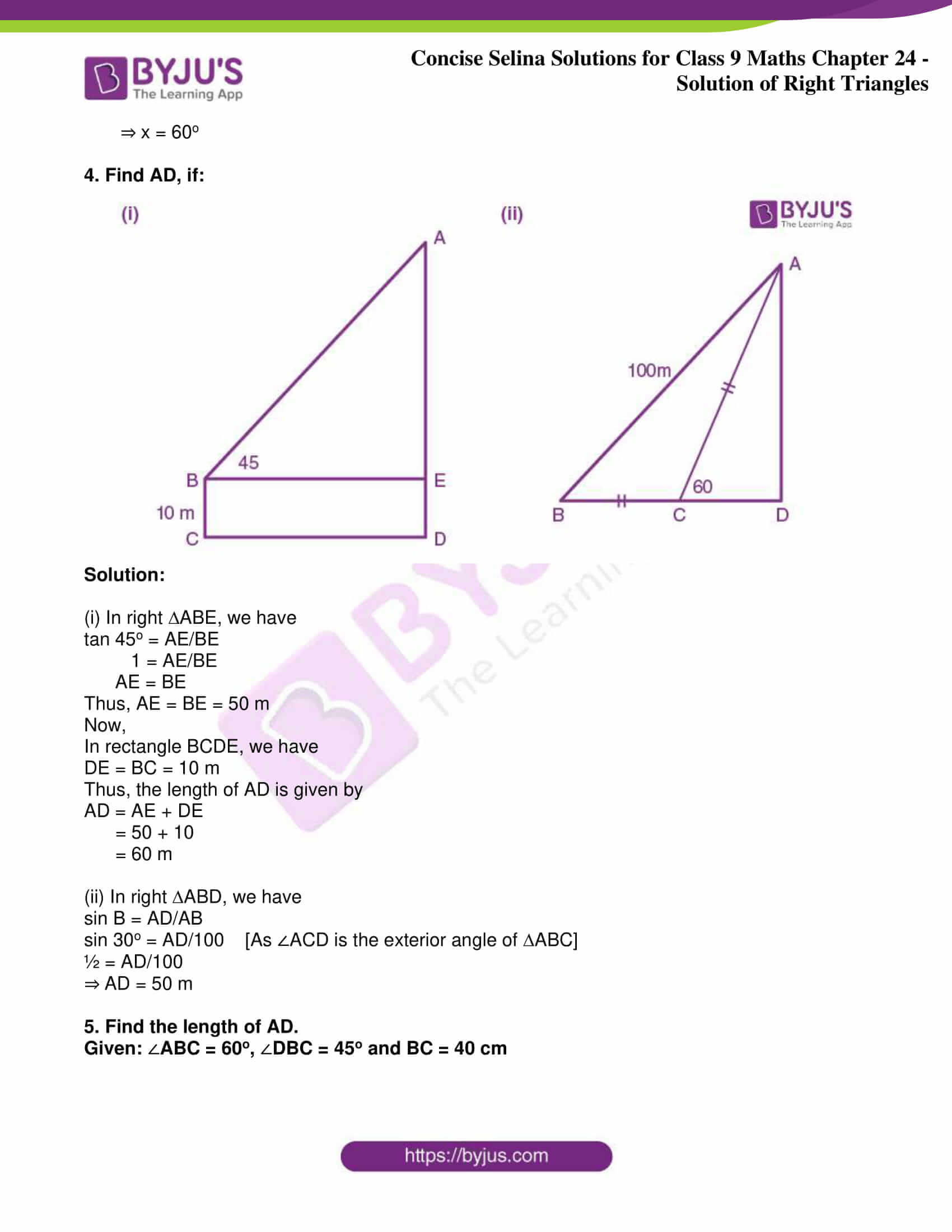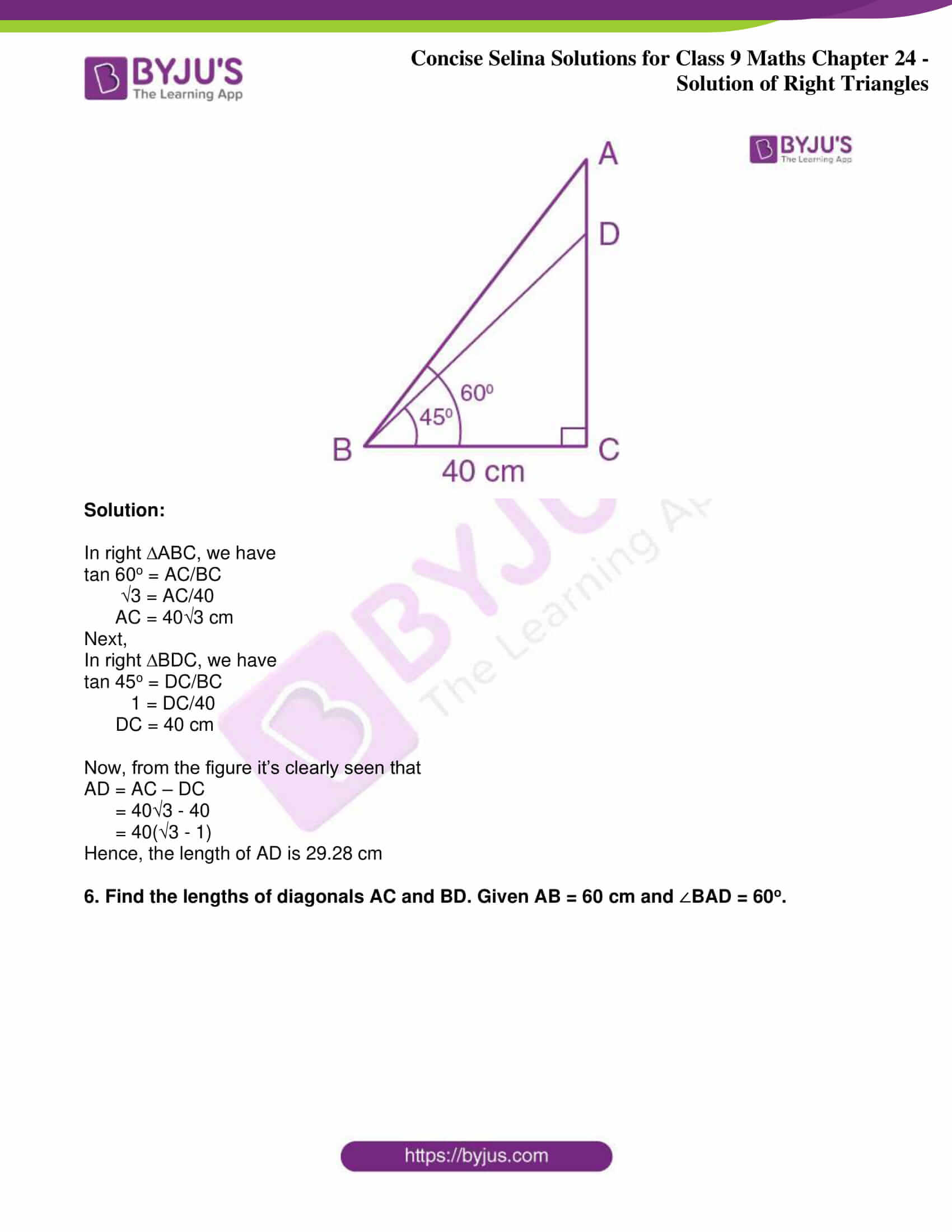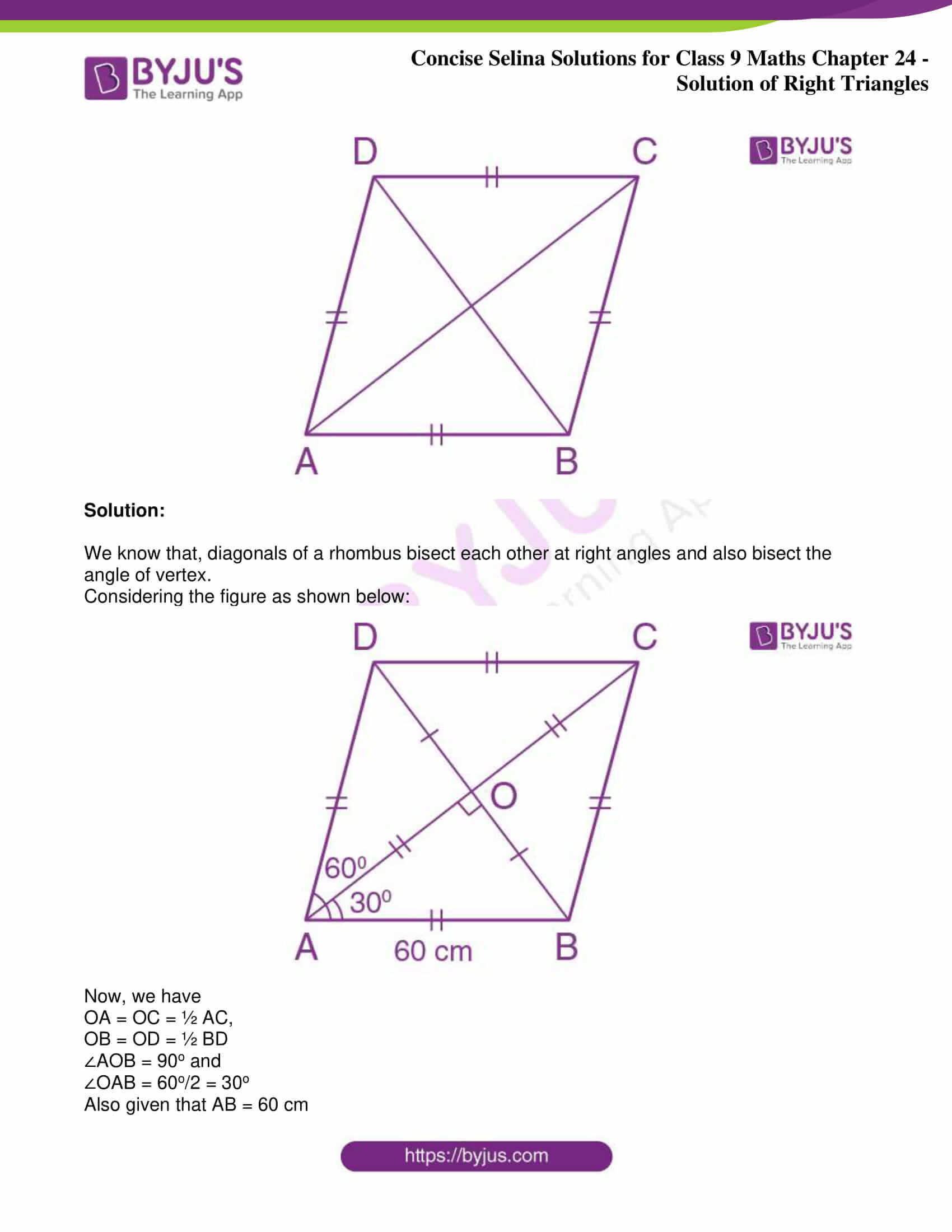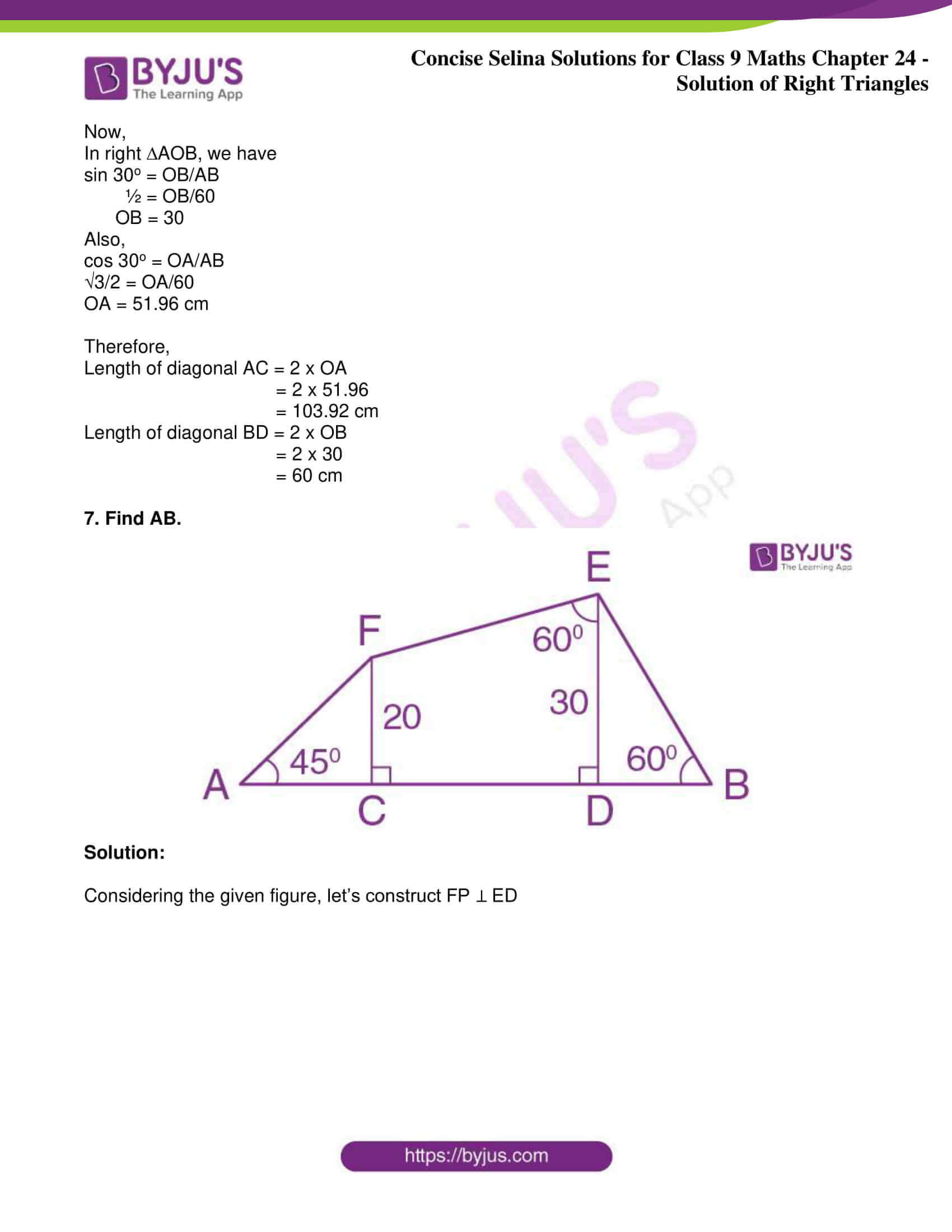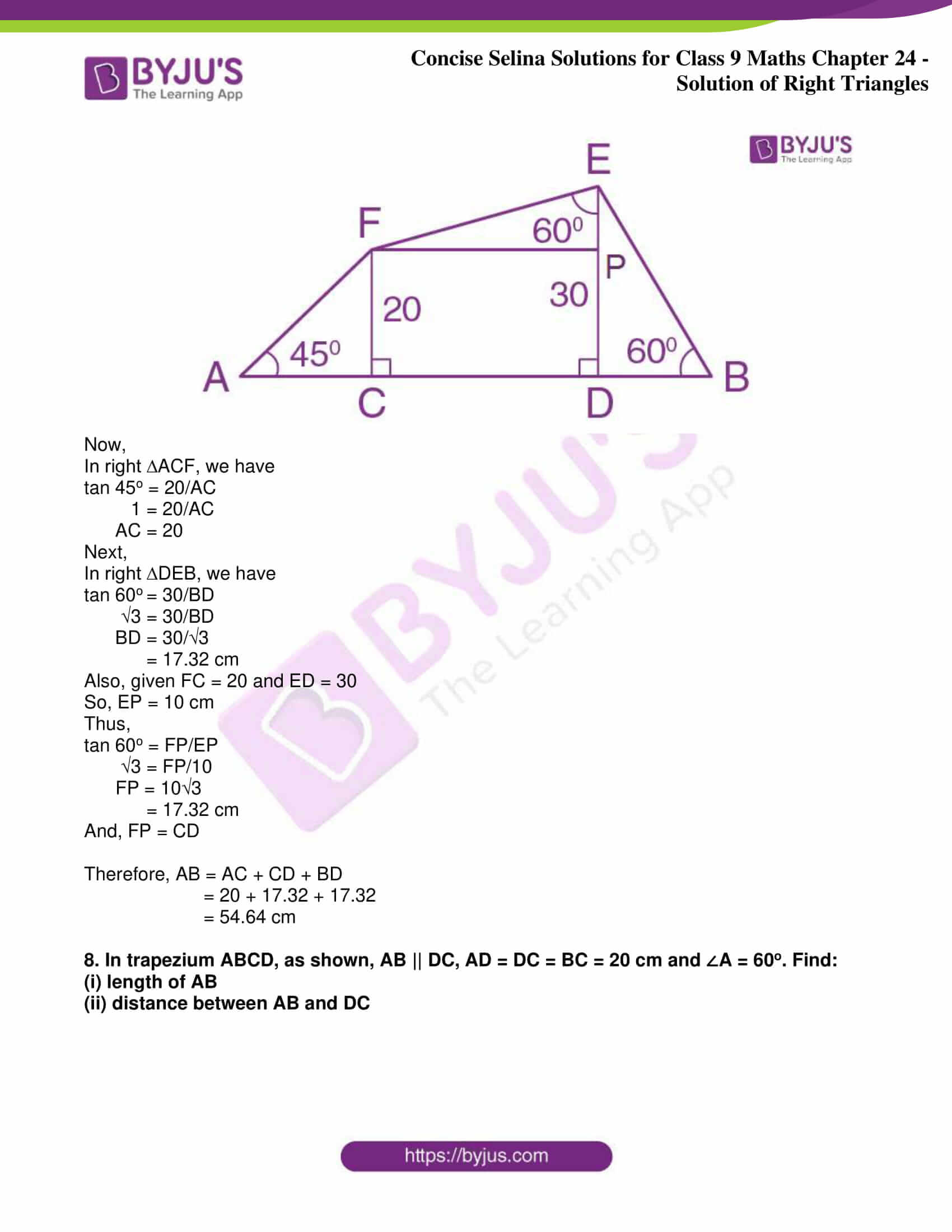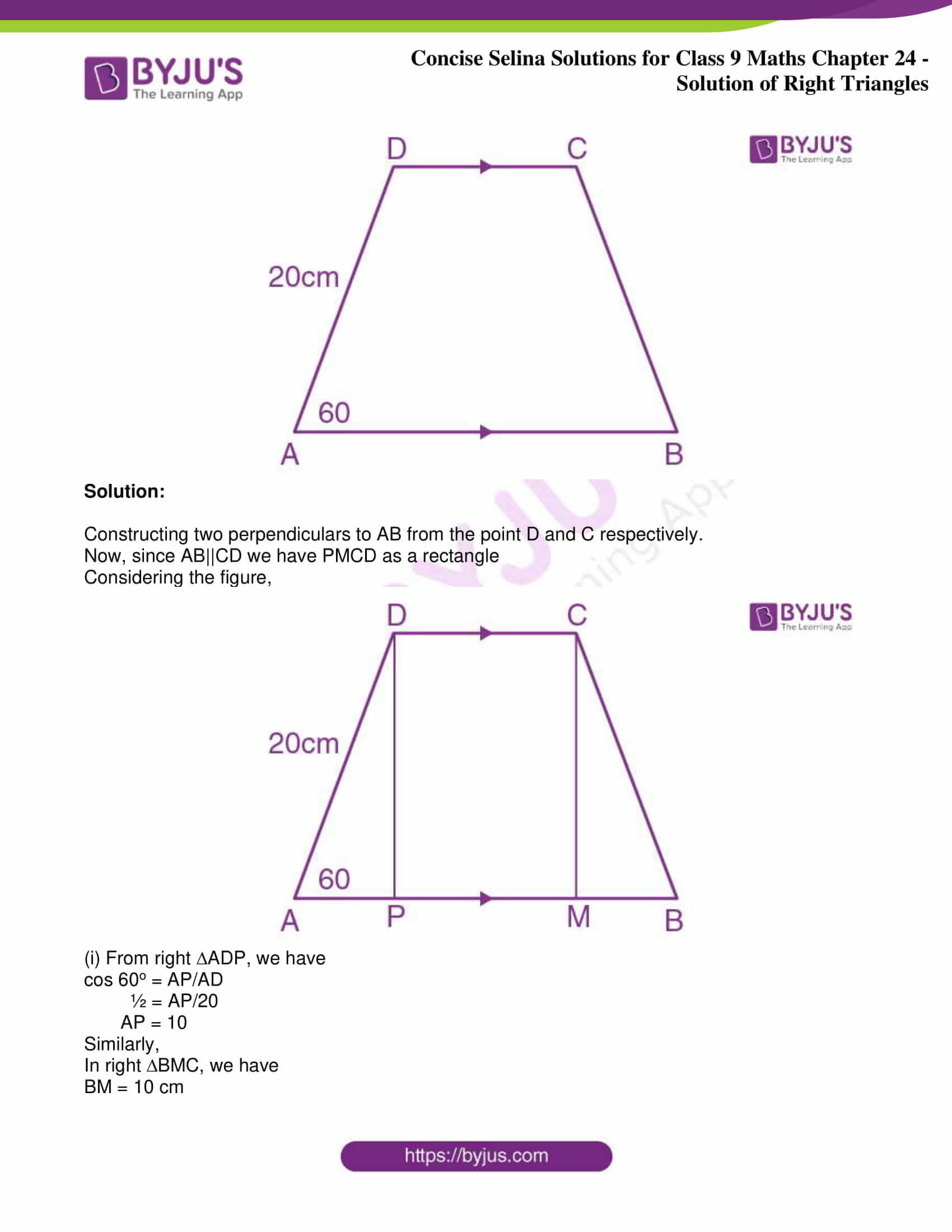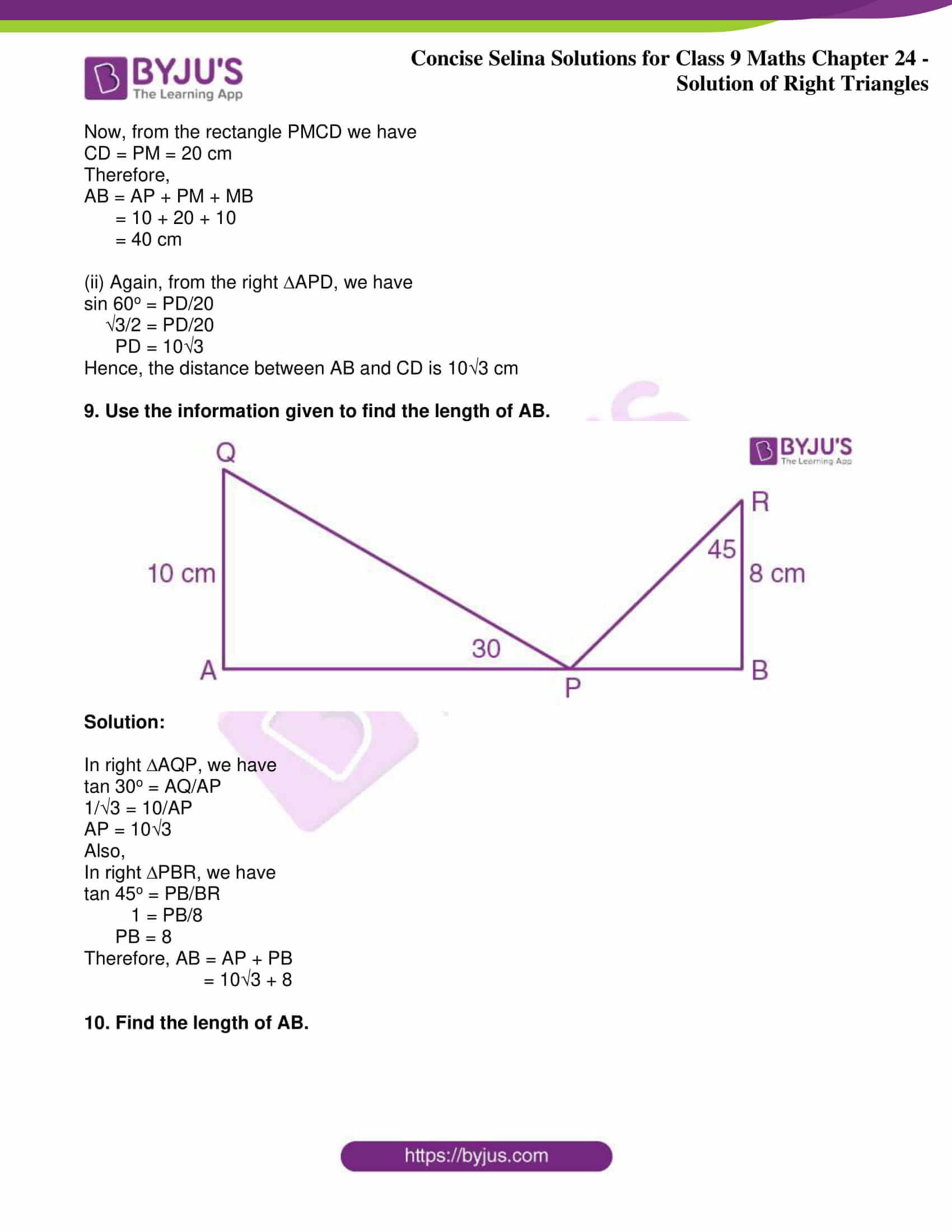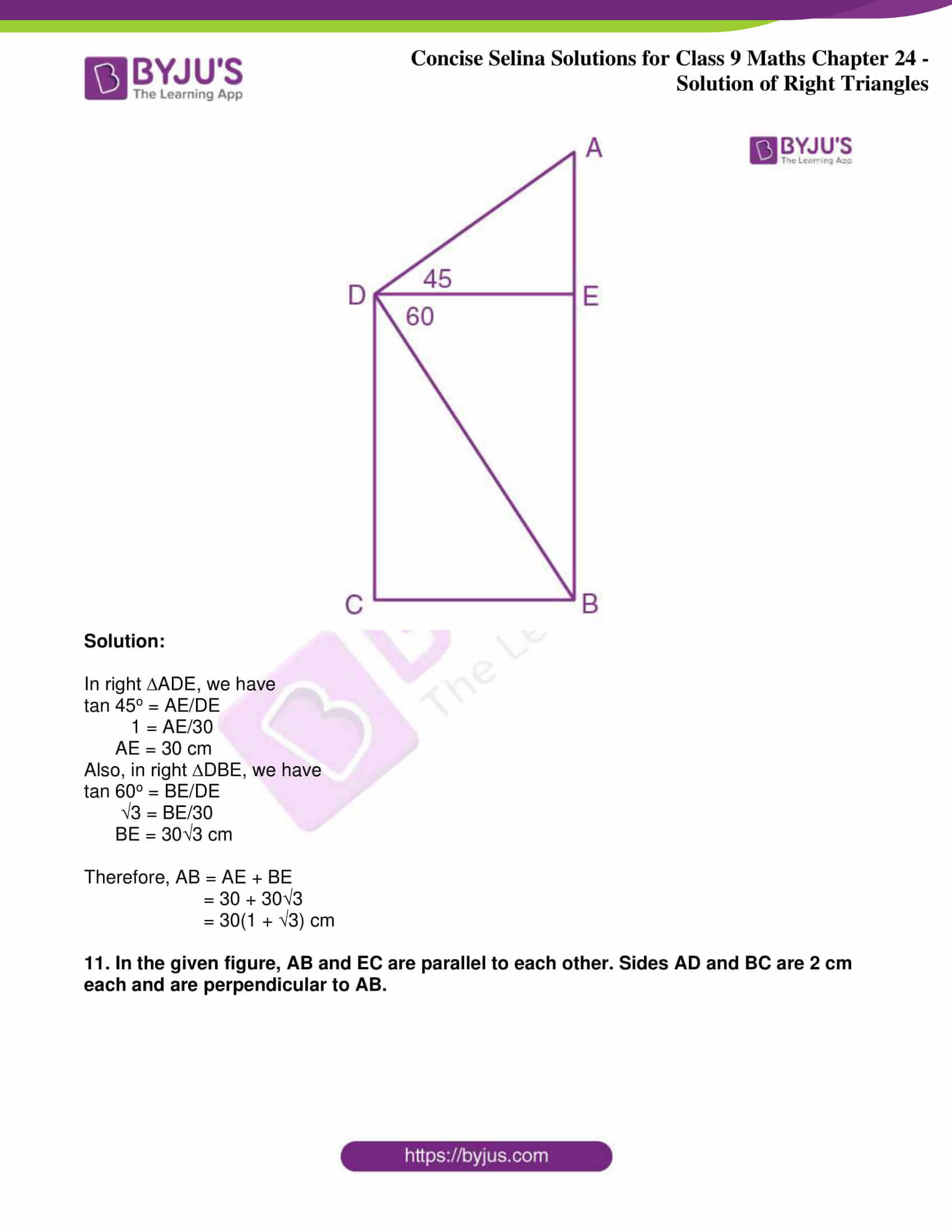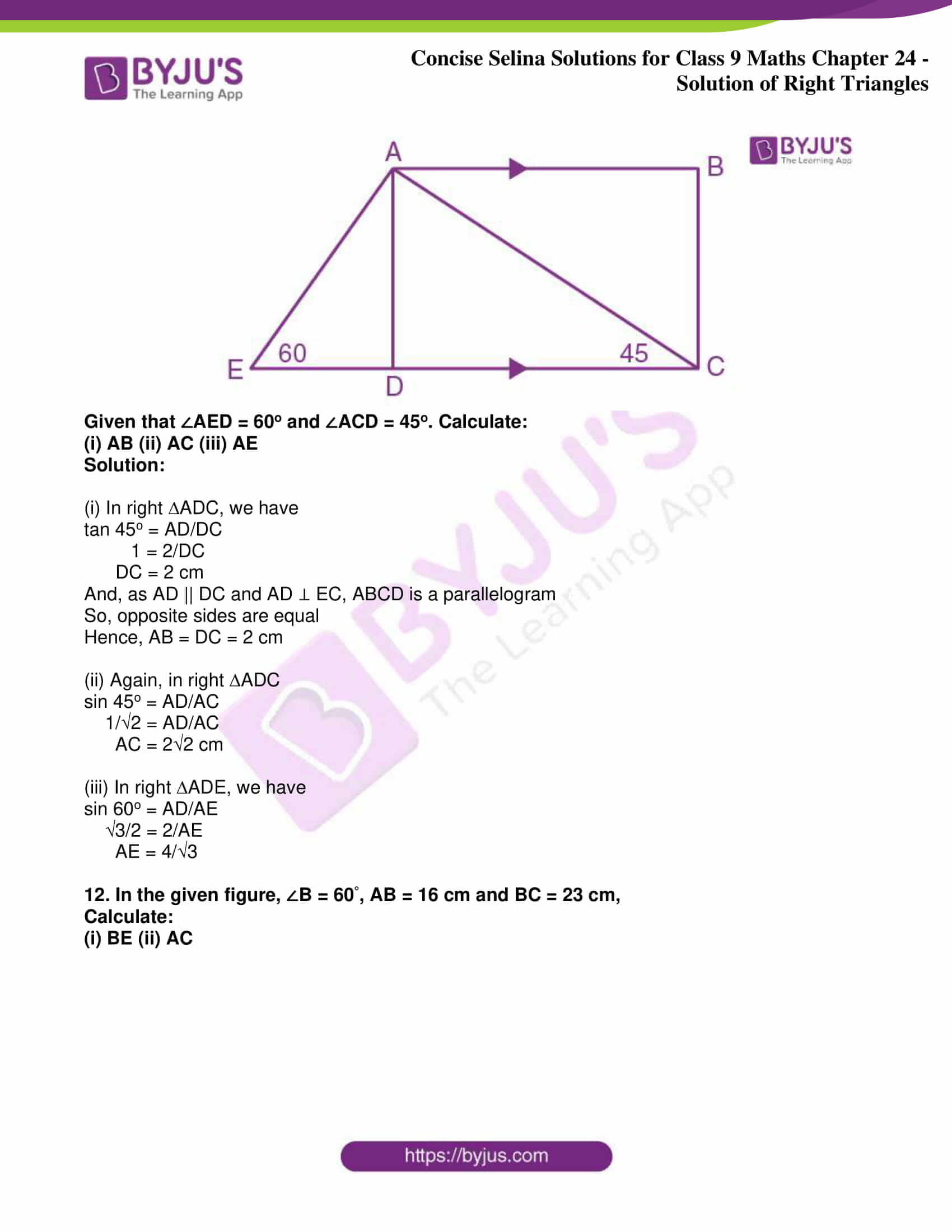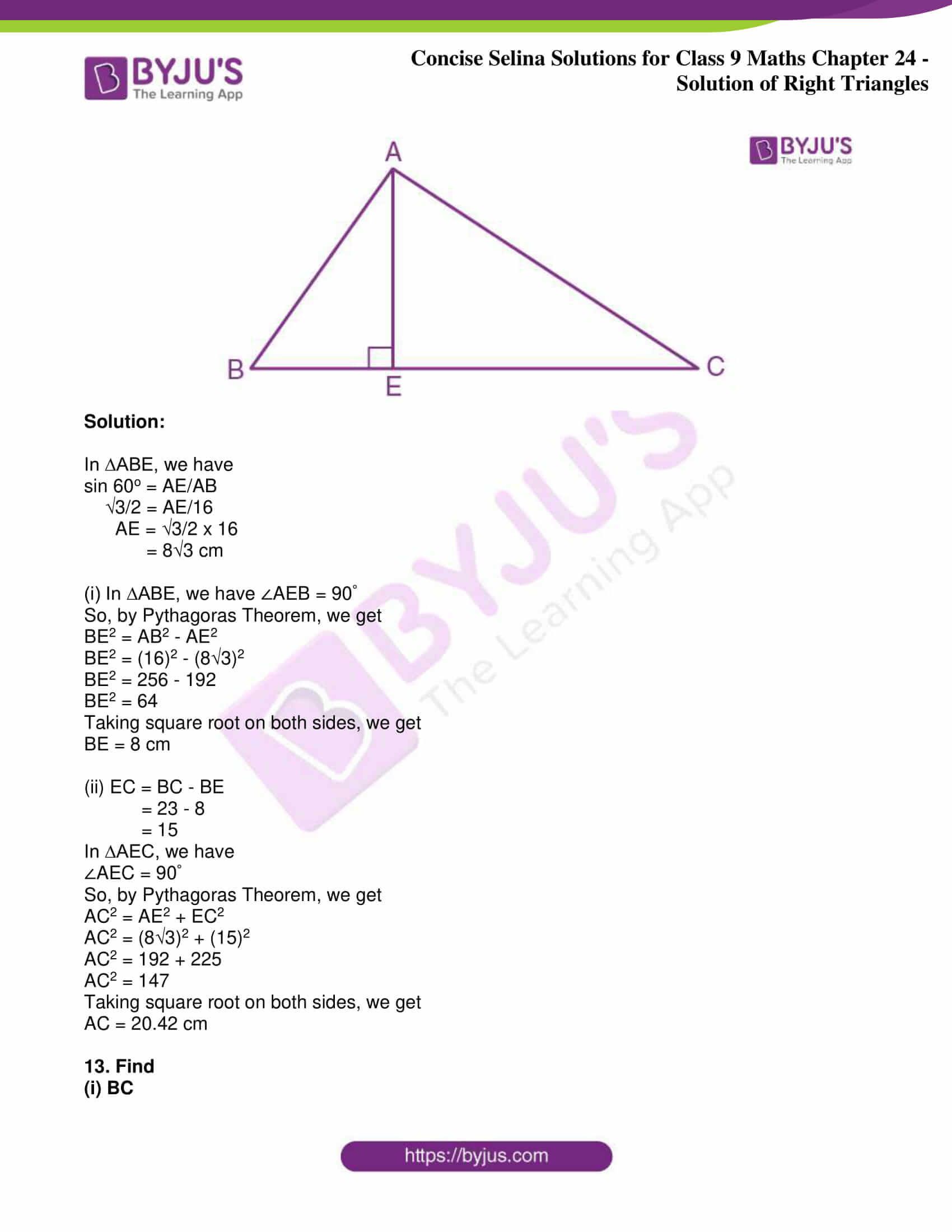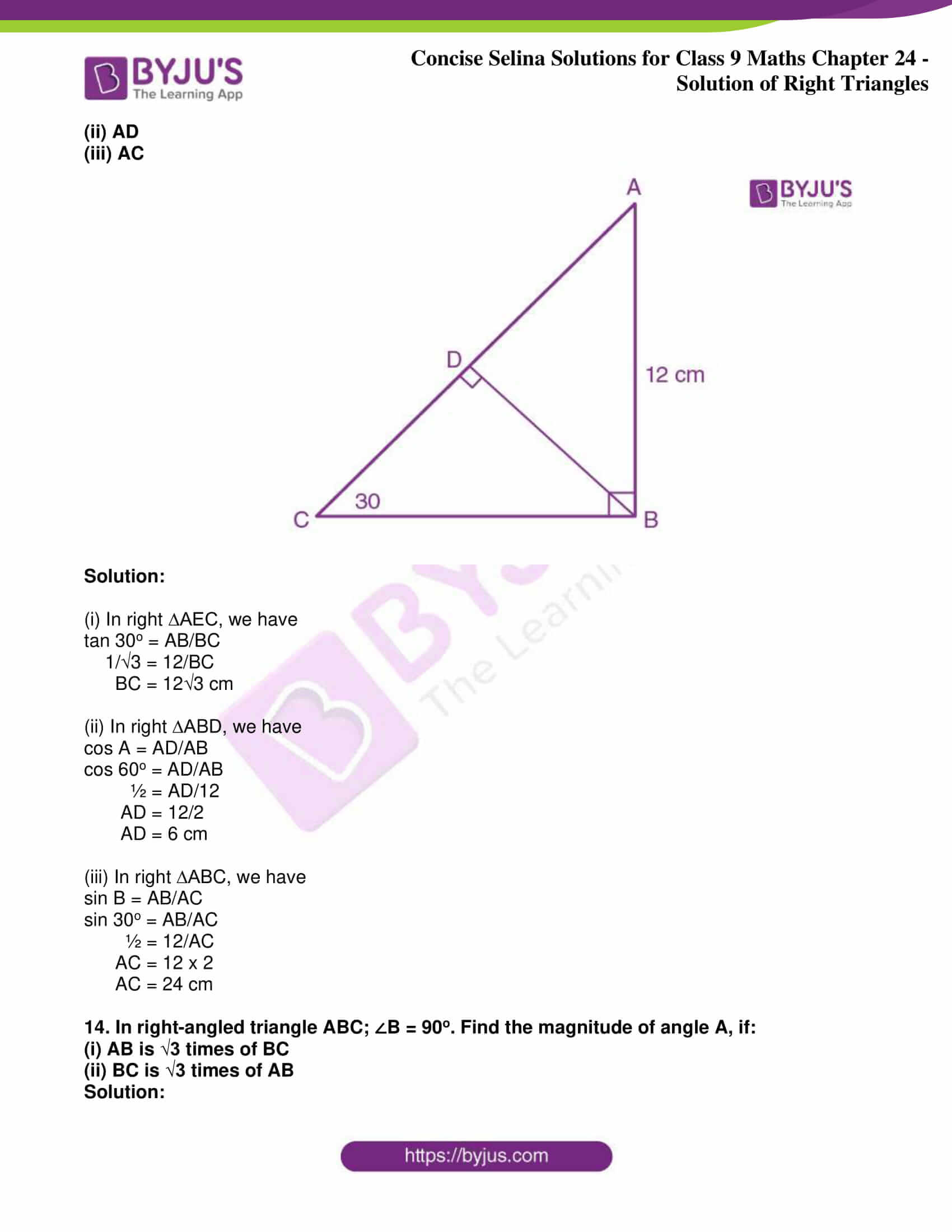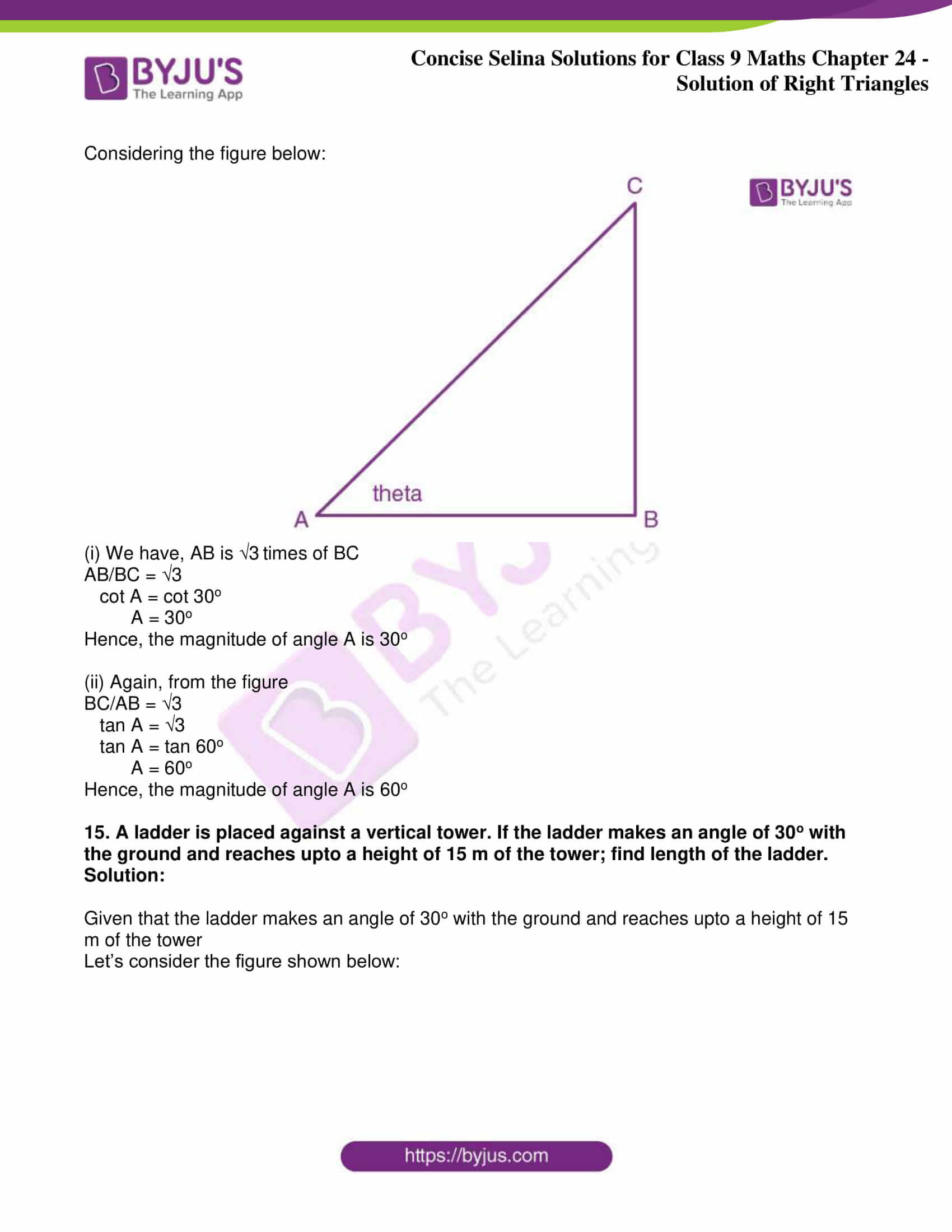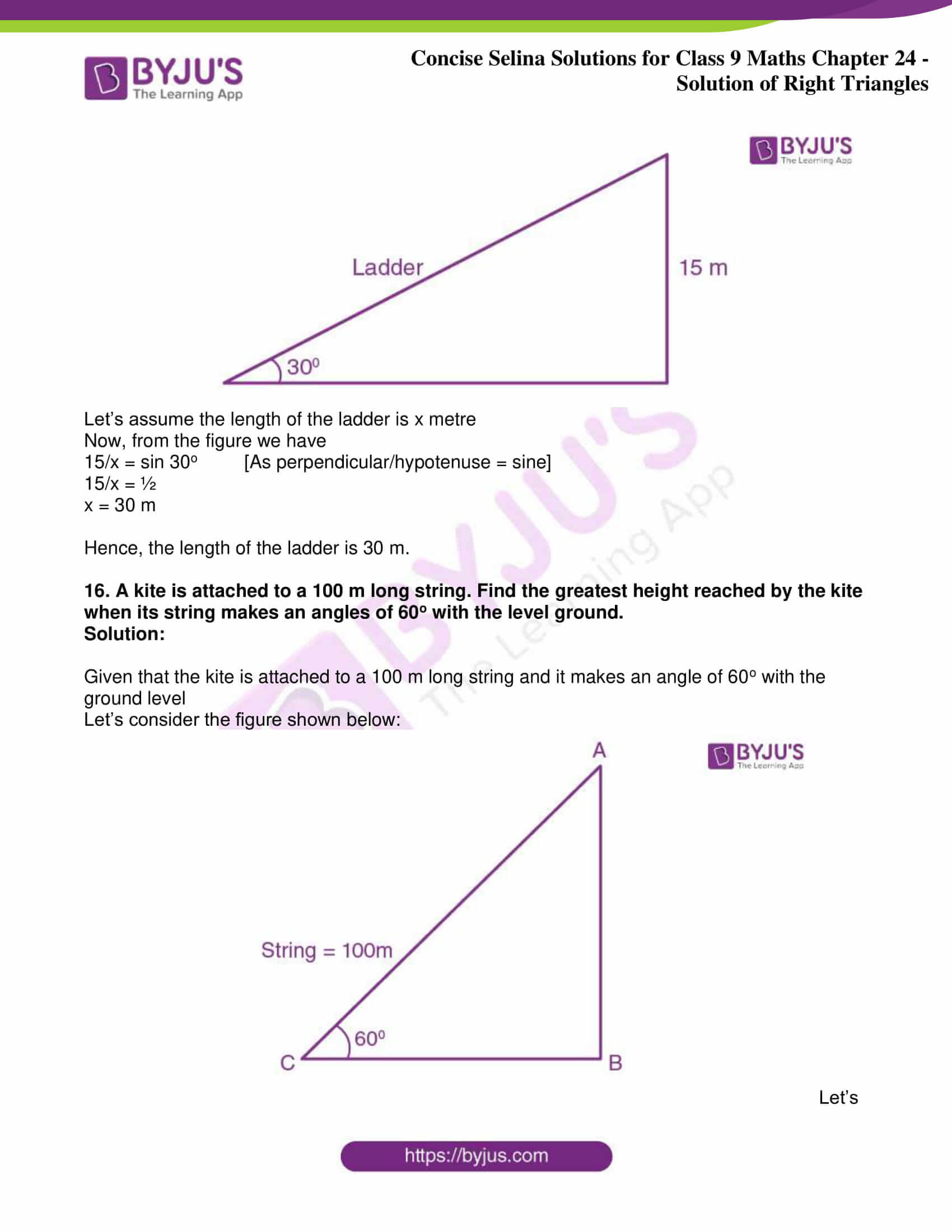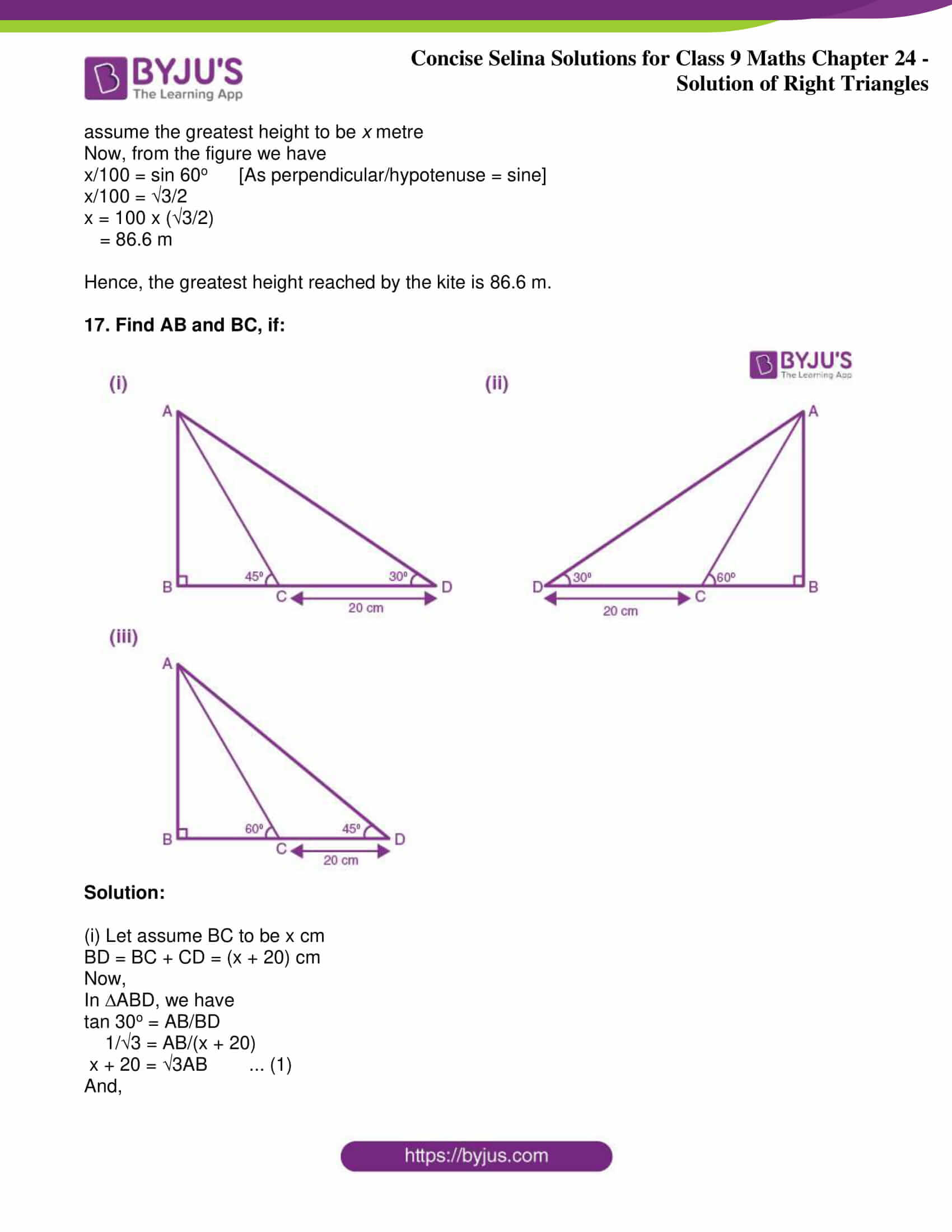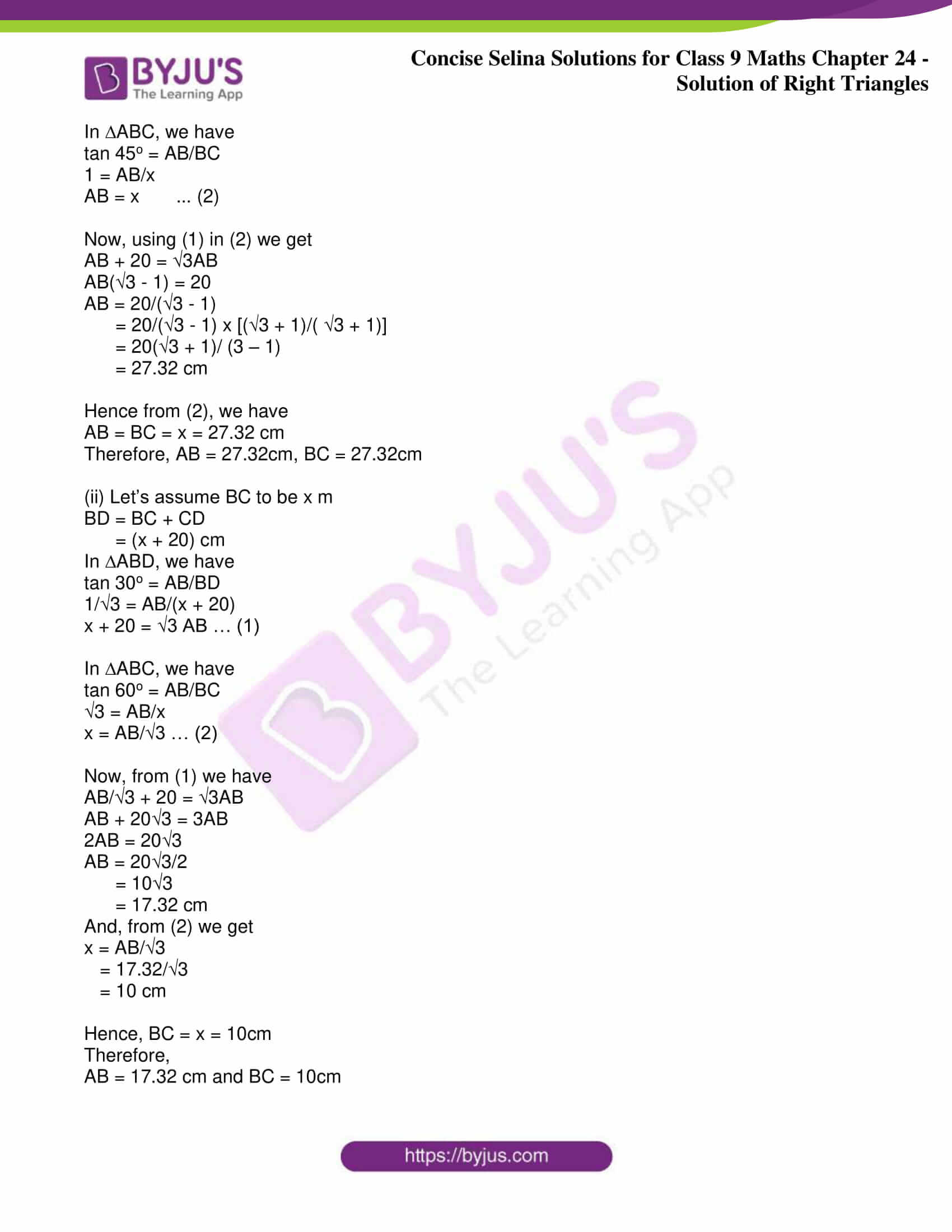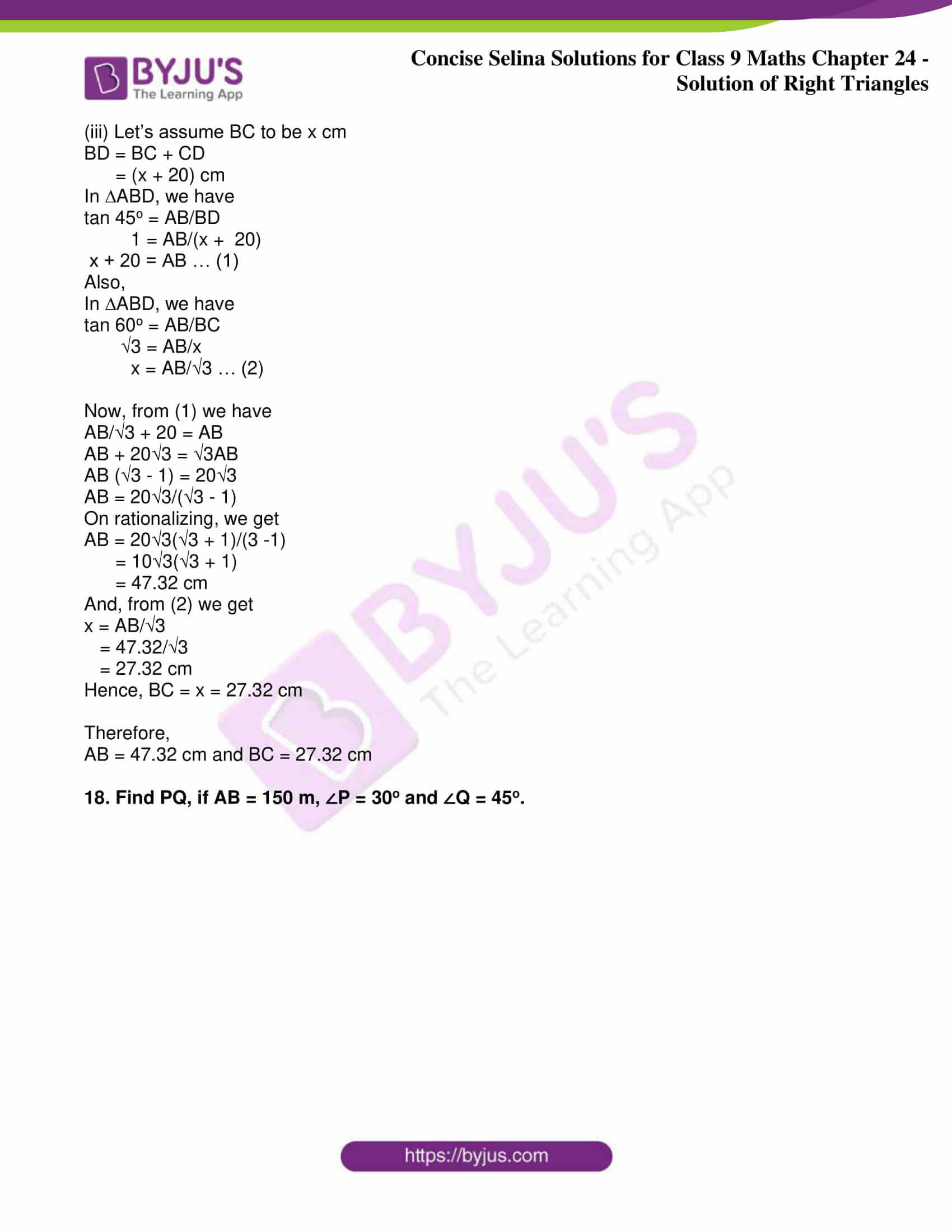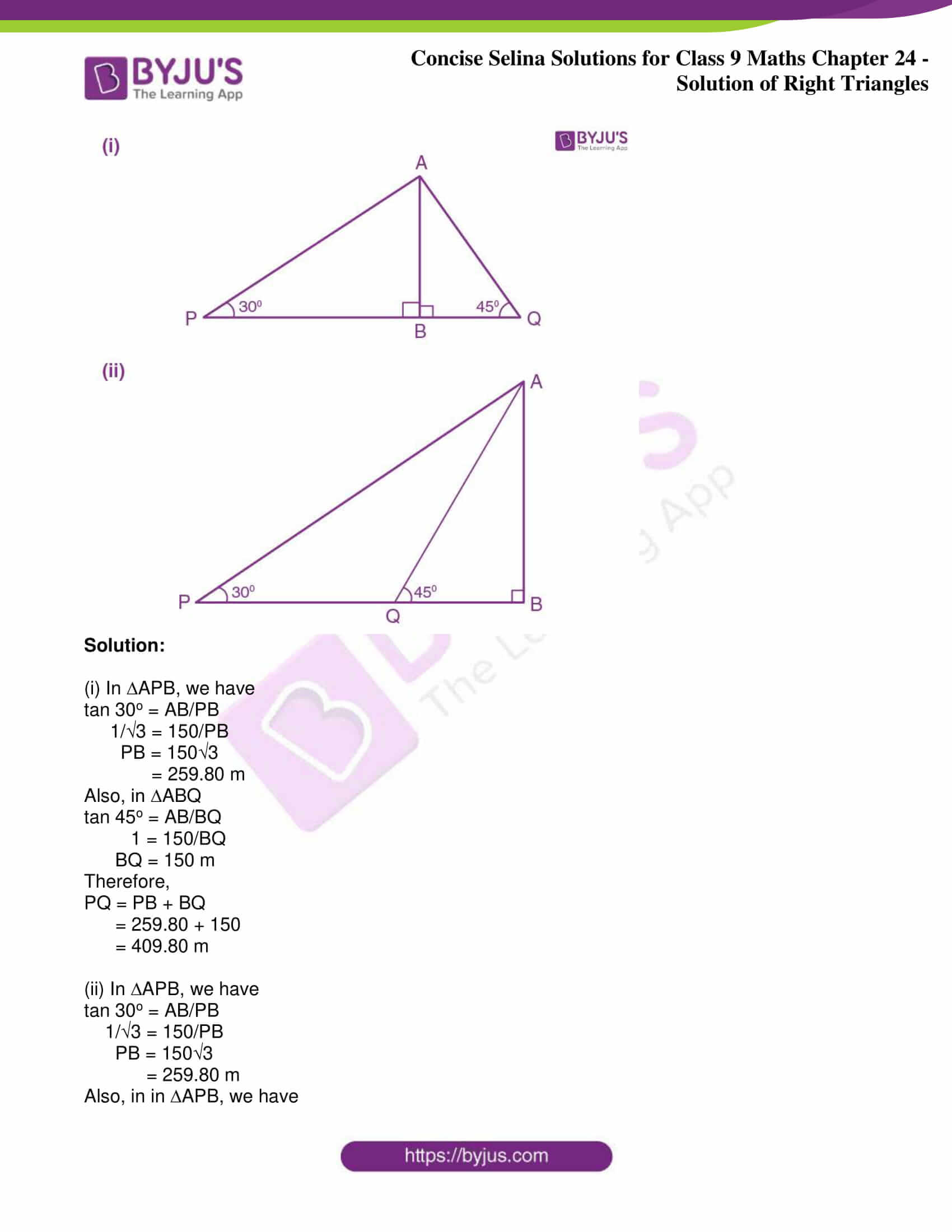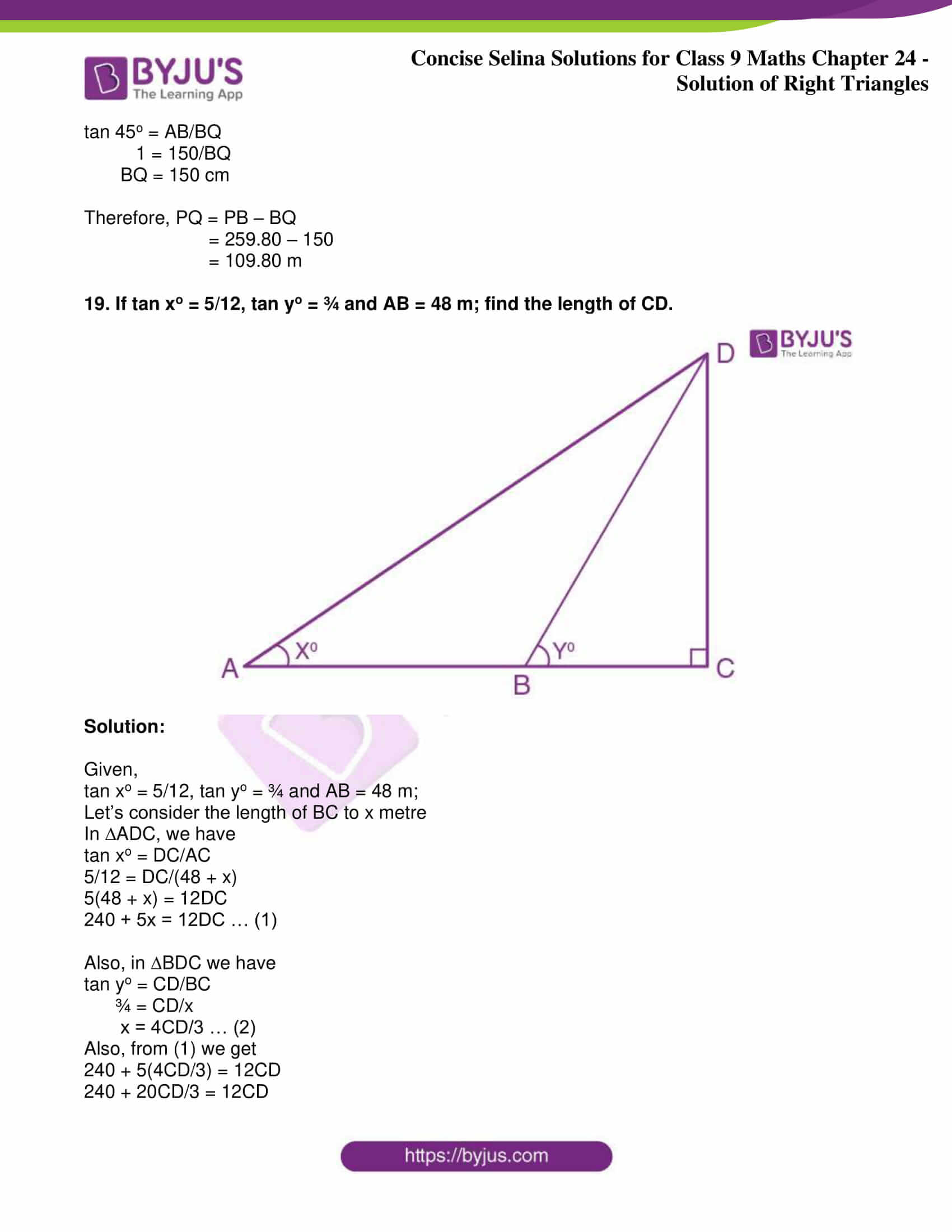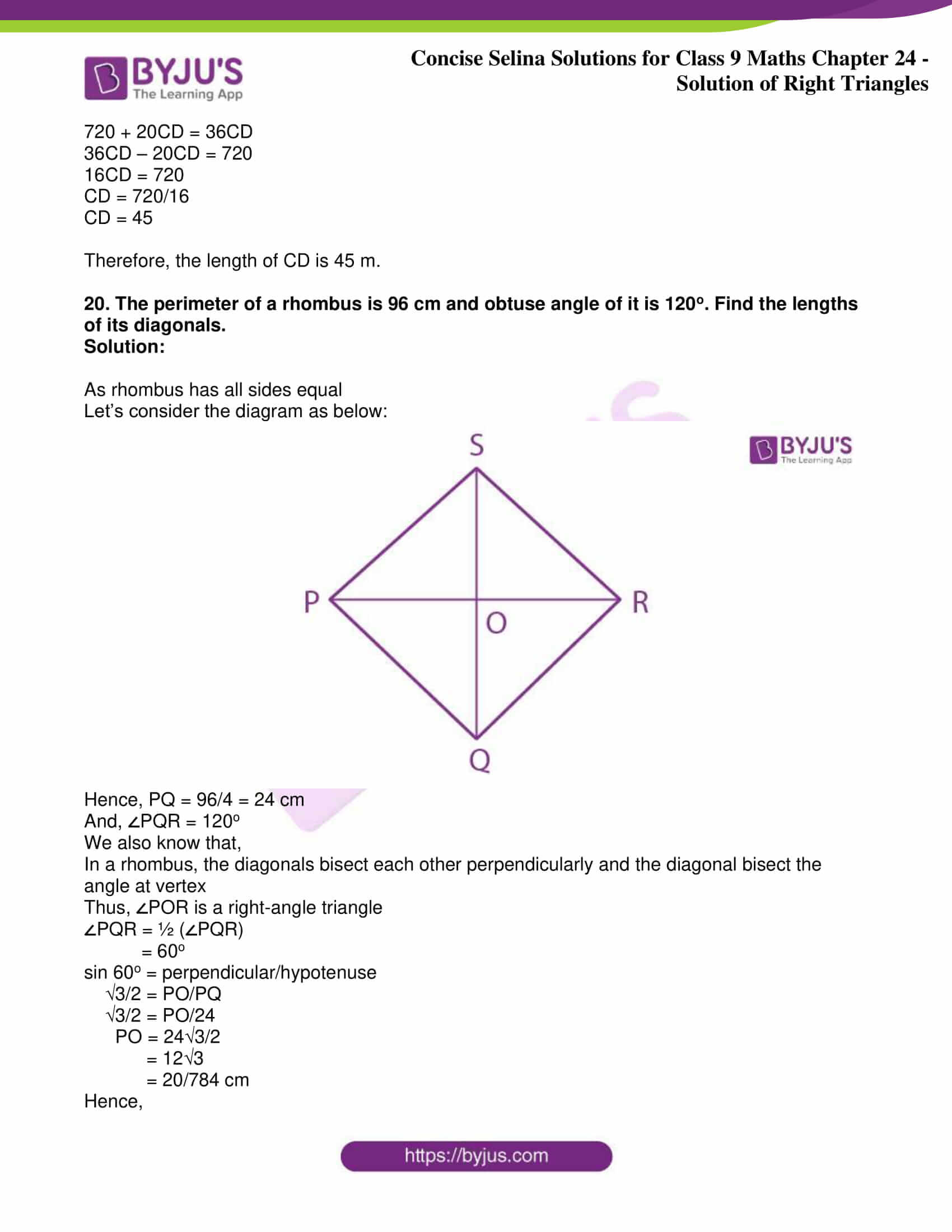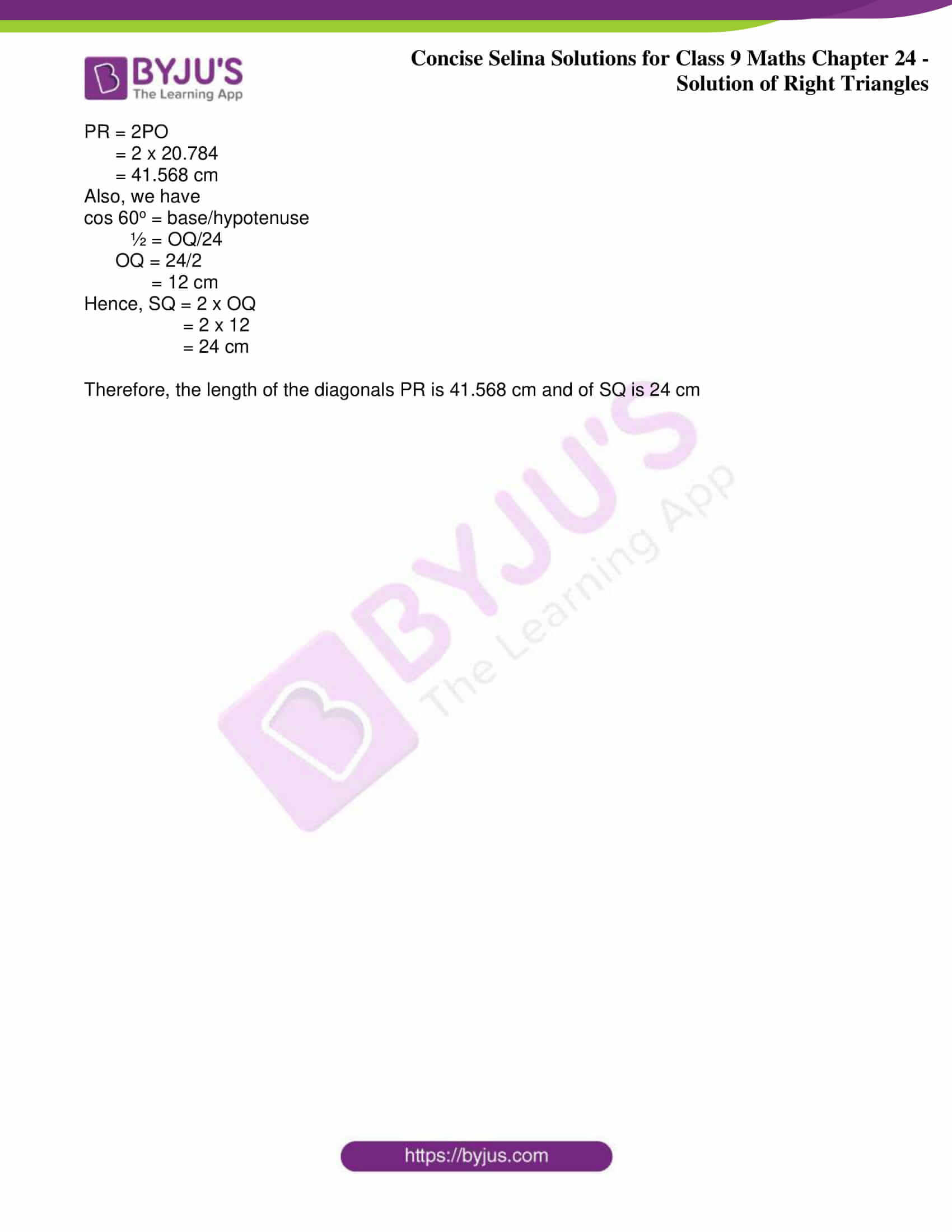Exercise 24

1. Find ‘x’ if: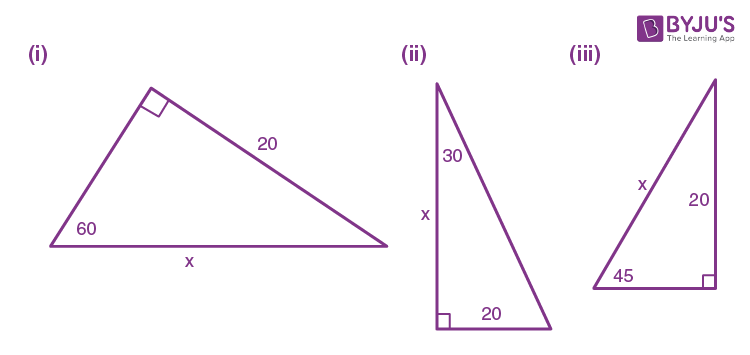Solution:

(i) From the figure, we have

sin 60o = 20/x

√3/2 = 20/x

∴ x = 40/√3

(ii) From the figure, we have

tan 30o = 20/x

1/√3 = 20/x

∴ x = 20√3

(iii) From the figure, we have

sin 45o = 20/x

1/√2 = 20/x

∴ x = 20√2

2. Find angle ‘A’ if: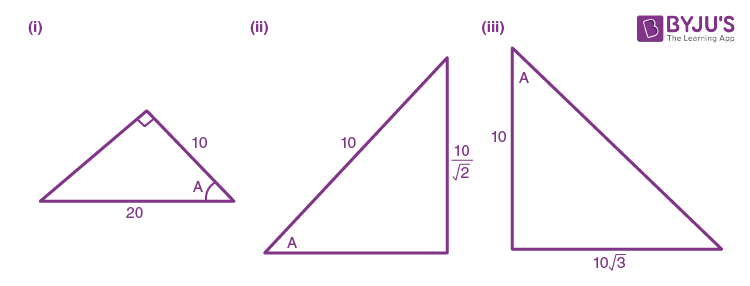Solution:

(i) From the figure, we have

cos A = 10/20

= ½

cos A = cos 60o

Hence,

A = 60o

(ii) From the figure, we have

sin A = (10/√2)/10

= 1/√2

sin A = sin 45o

Hence,

A = 45o

(iii) From the figure, we have

tan A = (10√3)/10

= √3

tan A = tan 60o

Hence,

A = 60o

3. Find angle ‘x’ if: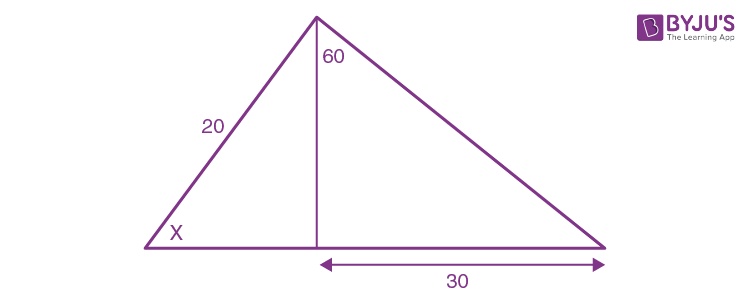Solution:

The given figure is drawn as follows: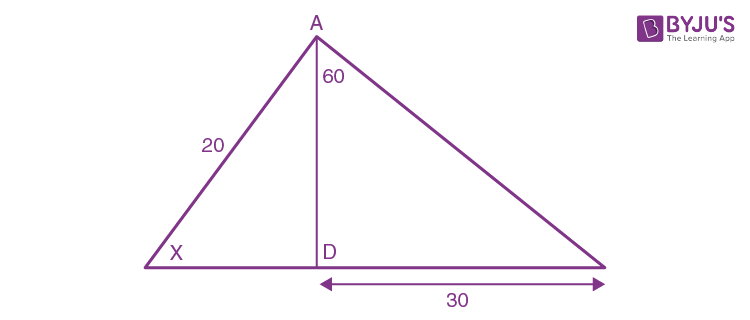We have,

Again,

Now,

20 sin x = 30/√3

sin x = 30/20√3

sin x = 3/2√3

sin x = √3/2

sin x = sin 60o

⇒ x = 60o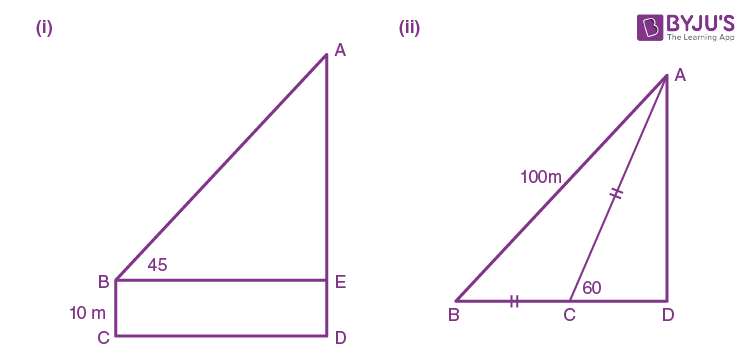Solution:

(i) In right ∆ABE, we have

tan 45o = AE/BE

1 = AE/BE

AE = BE

Thus, AE = BE = 50 m

Now,

In rectangle BCDE, we have

DE = BC = 10 m

Thus, the length of AD is given by

= 50 + 10

= 60 m

(ii) In right ∆ABD, we have

sin 30o = AD/100 [As ∠ACD is the exterior angle of ∆ABC]

5. Find the length of AD.

Given: ABC = 60o, DBC = 45o and BC = 40 cm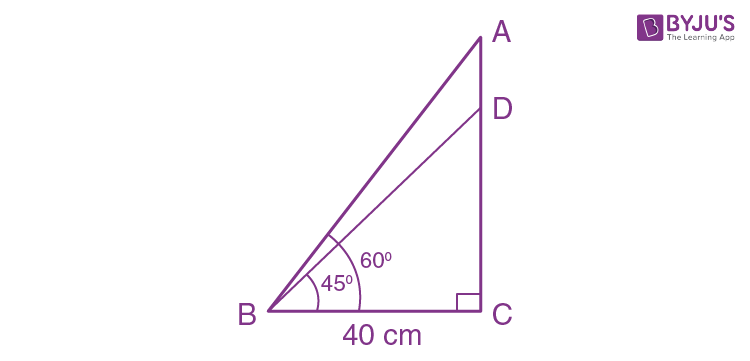Solution:

In right ∆ABC, we have

tan 60o = AC/BC

√3 = AC/40

AC = 40√3 cm

Next,

In right ∆BDC, we have

tan 45o = DC/BC

1 = DC/40

DC = 40 cm

Now, from the figure it’s clearly seen that

= 40√3 – 40

= 40(√3 – 1)

Hence, the length of AD is 29.28 cm

6. Find the lengths of diagonals AC and BD. Given AB = 60 cm and BAD = 60o.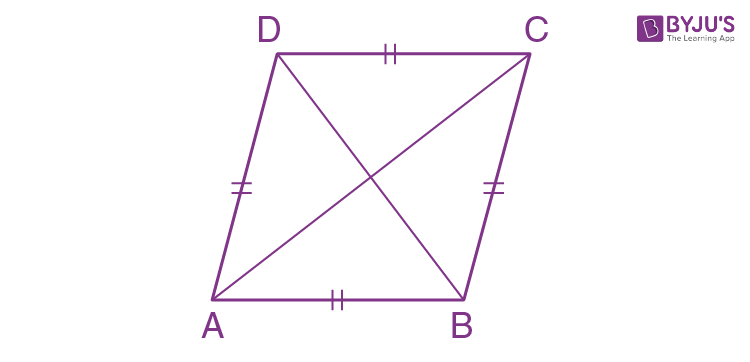Solution:

We know that, diagonals of a rhombus bisect each other at right angles and also bisect the angle of vertex.

Considering the figure as shown below: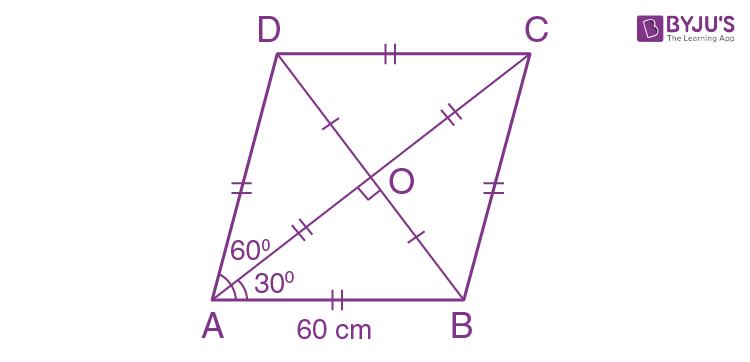Now, we have

OA = OC = ½ AC,

OB = OD = ½ BD

∠AOB = 90o and

∠OAB = 60o/2 = 30o

Also given that AB = 60 cm

Now,

In right ∆AOB, we have

sin 30o = OB/AB

½ = OB/60

OB = 30

Also,

cos 30o = OA/AB

√3/2 = OA/60

OA = 51.96 cm

Therefore,

Length of diagonal AC = 2 x OA

= 2 x 51.96

= 103.92 cm

Length of diagonal BD = 2 x OB

= 2 x 30

= 60 cm

7. Find AB.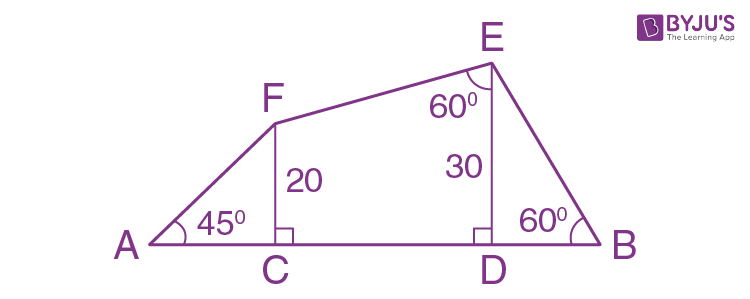Solution:

Considering the given figure, let’s construct FP ⊥ ED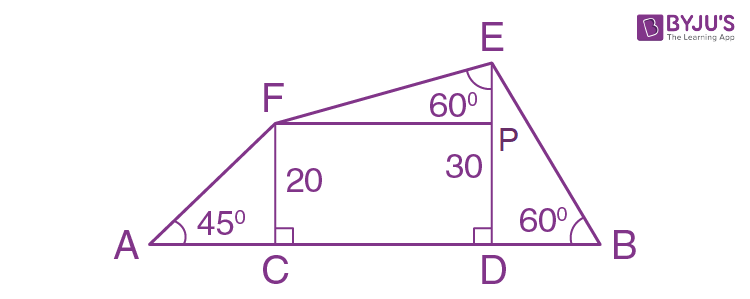Now,

In right ∆ACF, we have

tan 45o = 20/AC

1 = 20/AC

AC = 20

Next,

In right ∆DEB, we have

tan 60o = 30/BD

√3 = 30/BD

BD = 30/√3

= 17.32 cm

Also, given FC = 20 and ED = 30

So, EP = 10 cm

Thus,

tan 60o = FP/EP

√3 = FP/10

FP = 10√3

= 17.32 cm

And, FP = CD

Therefore, AB = AC + CD + BD

= 20 + 17.32 + 17.32

= 54.64 cm

8. In trapezium ABCD, as shown, AB || DC, AD = DC = BC = 20 cm and ∠A = 60o. Find:

(i) length of AB

(ii) distance between AB and DC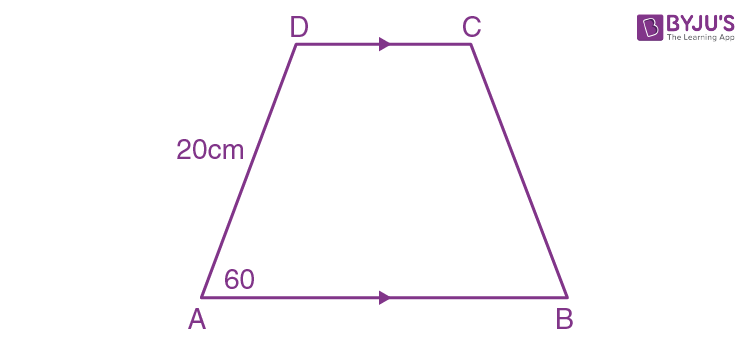Solution:

Constructing two perpendiculars to AB from the point D and C respectively.

Now, since AB||CD we have PMCD as a rectangle

Considering the figure,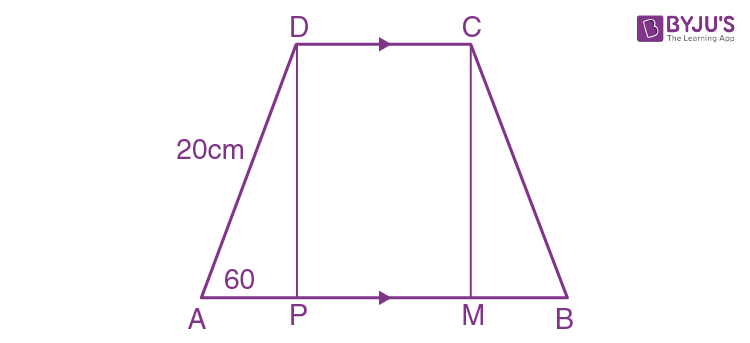(i) From right ∆ADP, we have

½ = AP/20

AP = 10

Similarly,

In right ∆BMC, we have

BM = 10 cm

Now, from the rectangle PMCD we have

CD = PM = 20 cm

Therefore,

AB = AP + PM + MB

= 10 + 20 + 10

= 40 cm

(ii) Again, from the right ∆APD, we have

sin 60o = PD/20

√3/2 = PD/20

PD = 10√3

Hence, the distance between AB and CD is 10√3 cm

9. Use the information given to find the length of AB.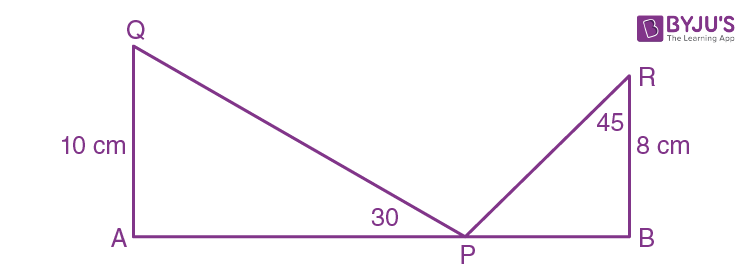Solution:

In right ∆AQP, we have

tan 30o = AQ/AP

1/√3 = 10/AP

AP = 10√3

Also,

In right ∆PBR, we have

tan 45o = PB/BR

1 = PB/8

PB = 8

Therefore, AB = AP + PB

= 10√3 + 8

10. Find the length of AB.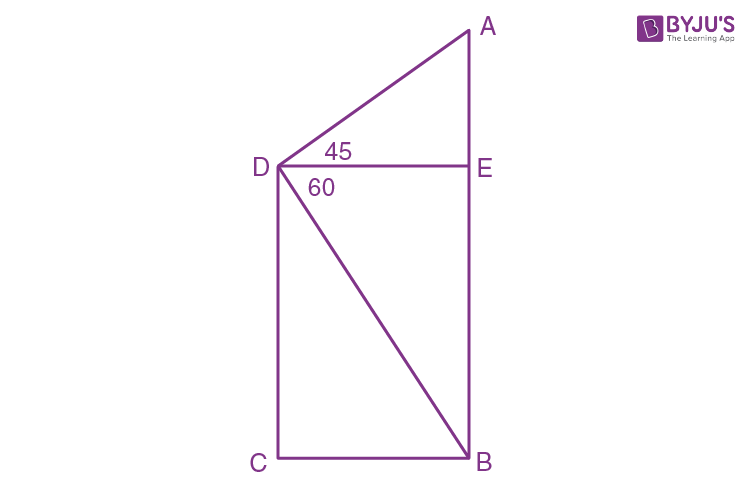Solution:

tan 45o = AE/DE

1 = AE/30

AE = 30 cm

Also, in right ∆DBE, we have

tan 60o = BE/DE

√3 = BE/30

BE = 30√3 cm

Therefore, AB = AE + BE

= 30 + 30√3

= 30(1 + √3) cm

11. In the given figure, AB and EC are parallel to each other. Sides AD and BC are 2 cm each and are perpendicular to AB.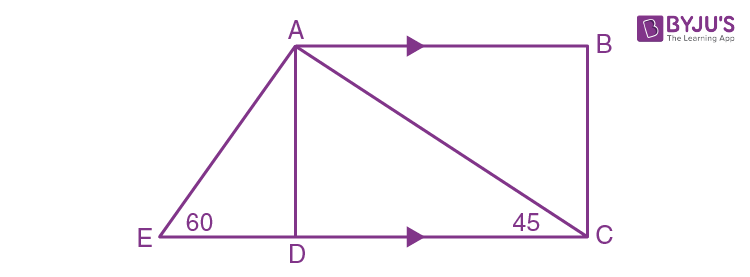Given that ∠AED = 60o and ∠ACD = 45o. Calculate:

(i) AB (ii) AC (iii) AE

Solution:

(i) In right ∆ADC, we have

1 = 2/DC

DC = 2 cm

And, as AD || DC and AD ⊥ EC, ABCD is a parallelogram

So, opposite sides are equal

Hence, AB = DC = 2 cm

AC = 2√2 cm

(iii) In right ∆ADE, we have

√3/2 = 2/AE

AE = 4/√3

12. In the given figure, ∠B = 60°, AB = 16 cm and BC = 23 cm,

Calculate:

(i) BE (ii) AC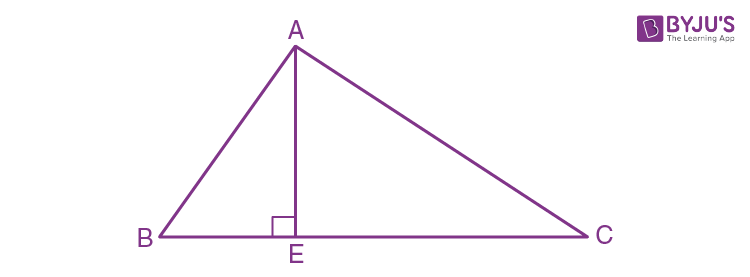Solution:

In ∆ABE, we have

sin 60o = AE/AB

√3/2 = AE/16

AE = √3/2 x 16

= 8√3 cm

(i) In ∆ABE, we have ∠AEB = 90°

So, by Pythagoras Theorem, we get

BE2 = AB2 – AE2

BE2 = (16)2 – (8√3)2

BE2 = 256 – 192

BE2 = 64

Taking square root on both sides, we get

BE = 8 cm

(ii) EC = BC – BE

= 23 – 8

= 15

In ∆AEC, we have

∠AEC = 90°

So, by Pythagoras Theorem, we get

AC2 = AE2 + EC2

AC2 = (8√3)2 + (15)2

AC2 = 192 + 225

AC2 = 147

Taking square root on both sides, we get

AC = 20.42 cm

13. Find

(i) BC

(iii) AC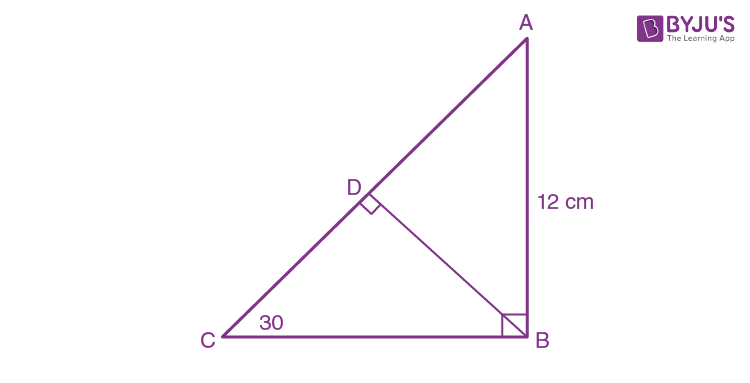Solution:

(i) In right ∆AEC, we have

tan 30o = AB/BC

1/√3 = 12/BC

BC = 12√3 cm

(ii) In right ∆ABD, we have

(iii) In right ∆ABC, we have

sin B = AB/AC

sin 30o = AB/AC

½ = 12/AC

AC = 12 x 2

AC = 24 cm

14. In right-angled triangle ABC; ∠B = 90o. Find the magnitude of angle A, if:

(i) AB is √3 times of BC

(ii) BC is √3 times of AB

Solution:

Considering the figure below: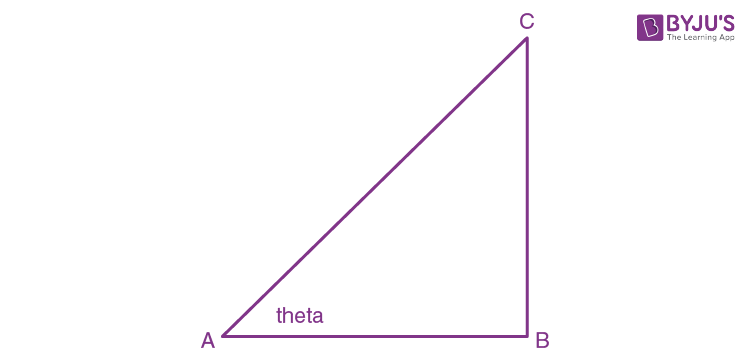(i) We have, AB is √3 times of BC

AB/BC = √3

cot A = cot 30o

A = 30o

Hence, the magnitude of angle A is 30o

(ii) Again, from the figure

BC/AB = √3

tan A = √3

tan A = tan 60o

A = 60o

Hence, the magnitude of angle A is 60o

15. A ladder is placed against a vertical tower. If the ladder makes an angle of 30o with the ground and reaches upto a height of 15 m of the tower; find length of the ladder.

Solution:

Given that the ladder makes an angle of 30o with the ground and reaches upto a height of 15 m of the tower

Let’s consider the figure shown below: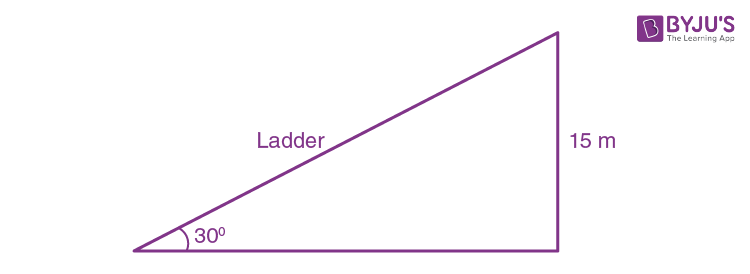Let’s assume the length of the ladder is x metre

Now, from the figure we have

15/x = sin 30o [As perpendicular/hypotenuse = sine]

15/x = ½

x = 30 m

Hence, the length of the ladder is 30 m.

16. A kite is attached to a 100 m long string. Find the greatest height reached by the kite when its string makes an angles of 60o with the level ground.

Solution:

Given that the kite is attached to a 100 m long string and it makes an angle of 60o with the ground level

Let’s consider the figure shown below: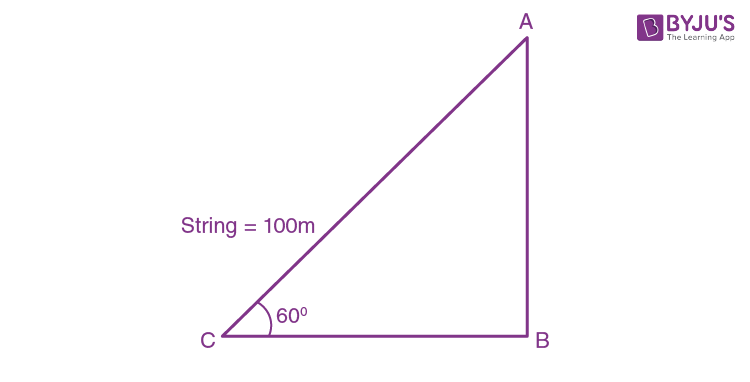Let’s assume the greatest height to be x metre

Now, from the figure we have

x/100 = sin 60o [As perpendicular/hypotenuse = sine]

x/100 = √3/2

x = 100 x (√3/2)

= 86.6 m

Hence, the greatest height reached by the kite is 86.6 m.

17. Find AB and BC, if: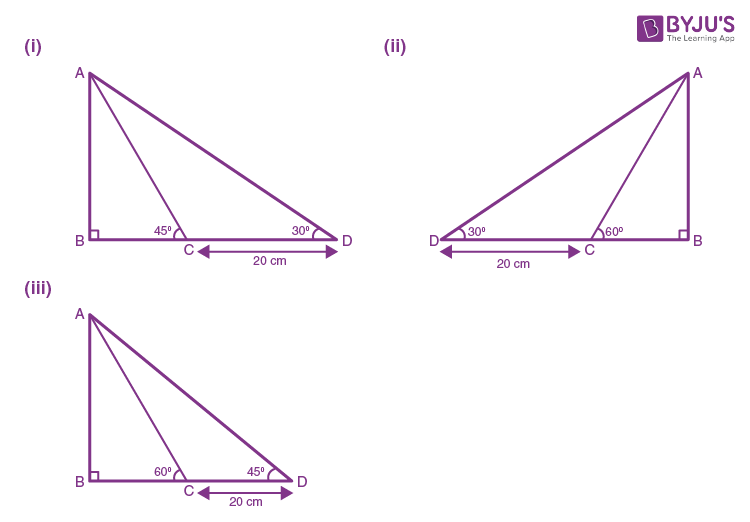Solution:

(i) Let assume BC to be x cm

BD = BC + CD = (x + 20) cm

Now,

In ∆ABD, we have

tan 30o = AB/BD

1/√3 = AB/(x + 20)

x + 20 = √3AB        … (1)

And,

In ∆ABC, we have

tan 45o = AB/BC

1 = AB/x

AB = x       … (2)

Now, using (1) in (2) we get

AB + 20 = √3AB

AB(√3 – 1) = 20

AB = 20/(√3 – 1)

= 20/(√3 – 1) x [(√3 + 1)/( √3 + 1)]

= 20(√3 + 1)/ (3 – 1)

= 27.32 cm

Hence from (2), we have

AB = BC = x = 27.32 cm

Therefore, AB = 27.32cm, BC = 27.32cm

(ii) Let’s assume BC to be x m

BD = BC + CD

= (x + 20) cm

In ∆ABD, we have

tan 30o = AB/BD

1/√3 = AB/(x + 20)

x + 20 = √3 AB … (1)

In ∆ABC, we have

tan 60o = AB/BC

√3 = AB/x

x = AB/√3 … (2)

Now, from (1) we have

AB/√3 + 20 = √3AB

AB + 20√3 = 3AB

2AB = 20√3

AB = 20√3/2

= 10√3

= 17.32 cm

And, from (2) we get

x = AB/√3

= 17.32/√3

= 10 cm

Hence, BC = x = 10cm

Therefore,

AB = 17.32 cm and BC = 10cm

(iii) Let’s assume BC to be x cm

BD = BC + CD

= (x + 20) cm

In ∆ABD, we have

tan 45o = AB/BD

1 = AB/(x + 20)

x + 20 = AB … (1)

Also,

In ∆ABD, we have

tan 60o = AB/BC

√3 = AB/x

x = AB/√3 … (2)

Now, from (1) we have

AB/√3 + 20 = AB

AB + 20√3 = √3AB

AB (√3 – 1) = 20√3

AB = 20√3/(√3 – 1)

On rationalizing, we get

AB = 20√3(√3 + 1)/(3 -1)

= 10√3(√3 + 1)

= 47.32 cm

And, from (2) we get

x = AB/√3

= 47.32/√3

= 27.32 cm

Hence, BC = x = 27.32 cm

Therefore,

AB = 47.32 cm and BC = 27.32 cm

18. Find PQ, if AB = 150 m, ∠P = 30o and ∠Q = 45o.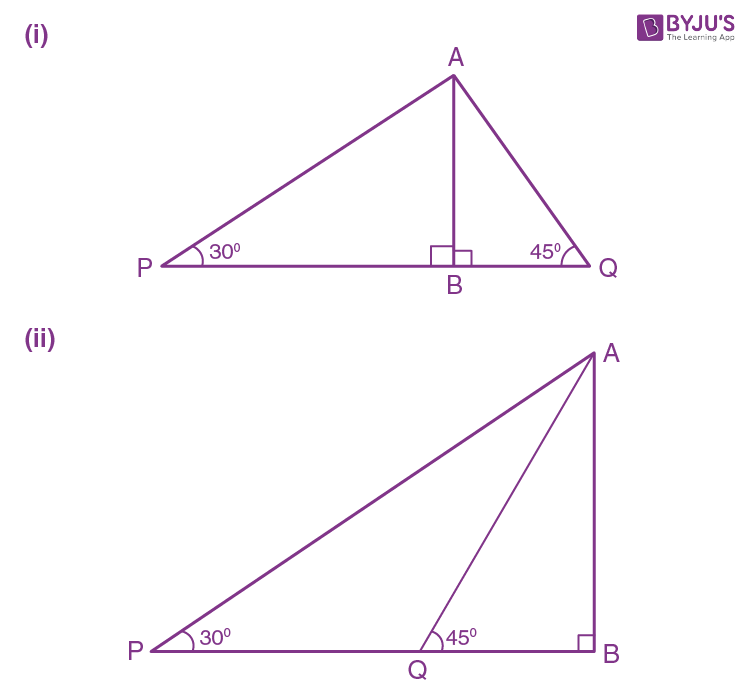Solution:

(i) In ∆APB, we have

tan 30o = AB/PB

1/√3 = 150/PB

PB = 150√3

= 259.80 m

Also, in ∆ABQ

tan 45o = AB/BQ

1 = 150/BQ

BQ = 150 m

Therefore,

PQ = PB + BQ

= 259.80 + 150

= 409.80 m

(ii) In ∆APB, we have

tan 30o = AB/PB

1/√3 = 150/PB

PB = 150√3

= 259.80 m

Also, in in ∆APB, we have

tan 45o = AB/BQ

1 = 150/BQ

BQ = 150 cm

Therefore, PQ = PB – BQ

= 259.80 – 150

= 109.80 m

19. If tan xo = 5/12, tan yo = ¾ and AB = 48 m; find the length of CD.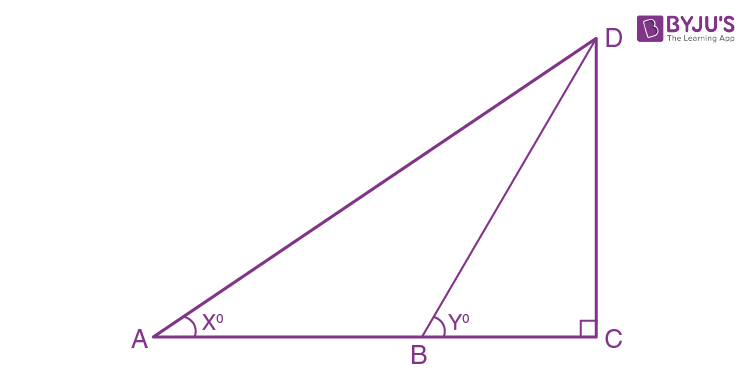Solution:

Given,

tan xo = 5/12, tan yo = ¾ and AB = 48 m;

Let’s consider the length of BC to x metre

tan xo = DC/AC

5/12 = DC/(48 + x)

5(48 + x) = 12DC

240 + 5x = 12DC … (1)

Also, in ∆BDC we have

tan yo = CD/BC

¾ = CD/x

x = 4CD/3 … (2)

Also, from (1) we get

240 + 5(4CD/3) = 12CD

240 + 20CD/3 = 12CD

720 + 20CD = 36CD

36CD – 20CD = 720

16CD = 720

CD = 720/16

CD = 45

Therefore, the length of CD is 45 m.

20. The perimeter of a rhombus is 96 cm and obtuse angle of it is 120o. Find the lengths of its diagonals.

Solution:

As rhombus has all sides equal

Let’s consider the diagram as below: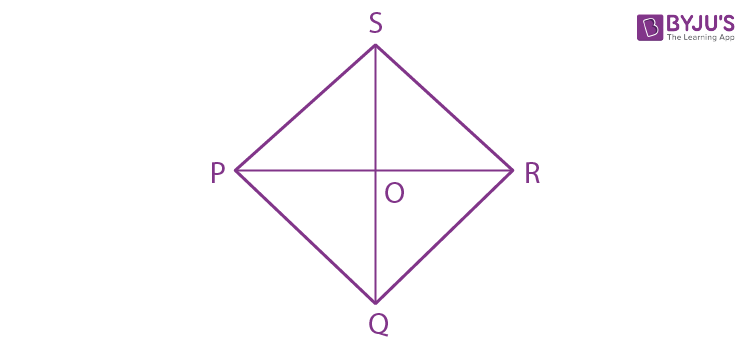Hence, PQ = 96/4 = 24 cm

And, PQR = 120o

We also know that,

In a rhombus, the diagonals bisect each other perpendicularly and the diagonal bisect the angle at vertex

Thus, POR is a right-angle triangle

PQR = ½ (PQR)

= 60o

sin 60o = perpendicular/hypotenuse

√3/2 = PO/PQ

√3/2 = PO/24

PO = 24√3/2

= 12√3

= 20/784 cm

Hence,

PR = 2PO

= 2 x 20.784

= 41.568 cm

Also, we have

cos 60o = base/hypotenuse

½ = OQ/24

OQ = 24/2

= 12 cm

Hence, SQ = 2 x OQ

= 2 x 12

= 24 cm

Therefore, the length of the diagonals PR is 41.568 cm and of SQ is 24 cm

## Selina Solutions for Class 9 Maths Chapter 24-Solution Of Right Triangles

The Chapter 24, Solution Of Right Triangles, contains 1 exercise and the Selina Solutions given here has the answers to all the questions present in these exercises. Let us have a look at some of the topics that are being discussed in this chapter.

24.1 Introduction

24.2 Simple 2-D problems involving one right angled triangle.

## Selina Solutions for Class 9 Maths Chapter 24-Solution Of Right Triangles

To solve a right angled triangle means, to find the values of remaining angles and remaining sides under two conditions, namely, when one side and one acute angle are given or when two sides of the triangle are given. Chapter 24 of class 9 gives the students an overview of the different problems related to the Solution Of Right Triangles. Read and learn Chapter 24 of Selina textbook to learn more about Solution Of Right Triangles along with the concepts covered in it. Solve the Selina Solutions for Class 9 effectively to score high in the examination.